# US8742704B2 - AC motor control device and AC motor driving system - Google Patents

## Info

Publication number
US8742704B2
US8742704B2 US13/202,667 US201013202667A US8742704B2 US 8742704 B2 US8742704 B2 US 8742704B2 US 201013202667 A US201013202667 A US 201013202667A US 8742704 B2 US8742704 B2 US 8742704B2
Authority
US
United States
Prior art keywords
motor
state
axis
axes
current
Prior art date
Legal status (The legal status is an assumption and is not a legal conclusion. Google has not performed a legal analysis and makes no representation as to the accuracy of the status listed.)
Active, expires
Application number
US13/202,667
Other versions
US20120007528A1 (en
Inventor
Junnosuke Nakatsugawa
Yoshitaka Iwaji
Norihisa IWASAKI
Hirokazu Nagura
Current Assignee (The listed assignees may be inaccurate. Google has not performed a legal analysis and makes no representation or warranty as to the accuracy of the list.)
Hitachi Ltd
Original Assignee
Hitachi Ltd
Priority date (The priority date is an assumption and is not a legal conclusion. Google has not performed a legal analysis and makes no representation as to the accuracy of the date listed.)
Filing date
Publication date
Priority to JP2009080853 priority Critical
Priority to JP2009-080853 priority
Application filed by Hitachi Ltd filed Critical Hitachi Ltd
Priority to PCT/JP2010/053123 priority patent/WO2010116815A1/en
Assigned to HITACHI, LTD. reassignment HITACHI, LTD. ASSIGNMENT OF ASSIGNORS INTEREST (SEE DOCUMENT FOR DETAILS). Assignors: NAGURA, HIROKAZU, IWAJI, YOSHITAKA, IWASAKI, NORIHISA, NAKATSUGAWA, JUNNOSUKE
Publication of US20120007528A1 publication Critical patent/US20120007528A1/en
Publication of US8742704B2 publication Critical patent/US8742704B2/en
Application granted granted Critical
Active legal-status Critical Current

• 230000004907 flux Effects 0.000 claims abstract description 154
• 230000000051 modifying Effects 0.000 claims description 36
• 238000004364 calculation methods Methods 0.000 claims description 34
• 230000001360 synchronised Effects 0.000 claims description 11
• 230000014509 gene expression Effects 0.000 claims description 8
• 239000010933 palladium Substances 0.000 claims 1
• 230000000875 corresponding Effects 0.000 abstract description 2
• 238000010586 diagram Methods 0.000 description 22
• 238000004458 analytical method Methods 0.000 description 11
• 238000001514 detection method Methods 0.000 description 11
• 238000006243 chemical reaction Methods 0.000 description 4
• 101700023868 YRDC Proteins 0.000 description 3
• 238000005516 engineering process Methods 0.000 description 3
• 101700031500 TECR Proteins 0.000 description 2
• 239000003990 capacitor Substances 0.000 description 2
• 230000003247 decreasing Effects 0.000 description 2
• 238000009499 grossing Methods 0.000 description 2
• 238000004450 types of analysis Methods 0.000 description 2
• 240000001439 Opuntia Species 0.000 description 1
• 235000020127 ayran Nutrition 0.000 description 1
• 238000004891 communication Methods 0.000 description 1
• 230000000694 effects Effects 0.000 description 1
• 230000001939 inductive effect Effects 0.000 description 1
• 230000003993 interaction Effects 0.000 description 1
• 229910000529 magnetic ferrite Inorganic materials 0.000 description 1
• 238000000034 method Methods 0.000 description 1
• 230000001629 suppression Effects 0.000 description 1
• 238000004804 winding Methods 0.000 description 1
• 229910000859 α-Fe Inorganic materials 0.000 description 1

## Images

•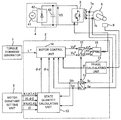•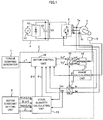•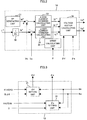•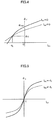•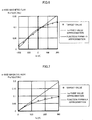•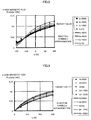•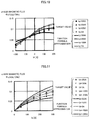•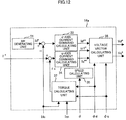•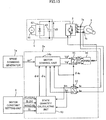•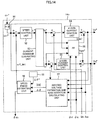•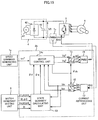•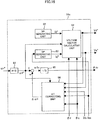•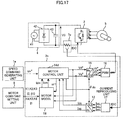•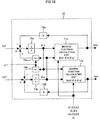•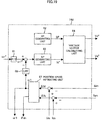•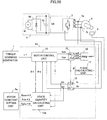•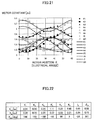•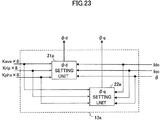•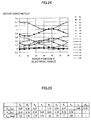•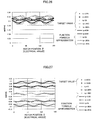•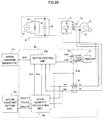•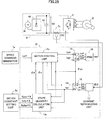•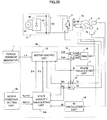•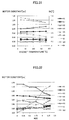•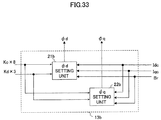•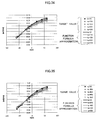•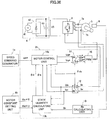•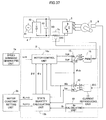•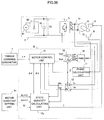•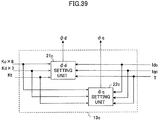## Classifications

• HELECTRICITY
• H02GENERATION; CONVERSION OR DISTRIBUTION OF ELECTRIC POWER
• H02PCONTROL OR REGULATION OF ELECTRIC MOTORS, ELECTRIC GENERATORS OR DYNAMO-ELECTRIC CONVERTERS; CONTROLLING TRANSFORMERS, REACTORS OR CHOKE COILS
• H02P21/00Arrangements or methods for the control of electric machines by vector control, e.g. by control of field orientation
• H02P21/14Estimation or adaptation of machine parameters, e.g. flux, current or voltage
• H02P21/141Flux estimation
• HELECTRICITY
• H02GENERATION; CONVERSION OR DISTRIBUTION OF ELECTRIC POWER
• H02PCONTROL OR REGULATION OF ELECTRIC MOTORS, ELECTRIC GENERATORS OR DYNAMO-ELECTRIC CONVERTERS; CONTROLLING TRANSFORMERS, REACTORS OR CHOKE COILS
• H02P21/00Arrangements or methods for the control of electric machines by vector control, e.g. by control of field orientation
• H02P21/14Estimation or adaptation of machine parameters, e.g. flux, current or voltage
• H02P21/18Estimation of position or speed
• HELECTRICITY
• H02GENERATION; CONVERSION OR DISTRIBUTION OF ELECTRIC POWER
• H02PCONTROL OR REGULATION OF ELECTRIC MOTORS, ELECTRIC GENERATORS OR DYNAMO-ELECTRIC CONVERTERS; CONTROLLING TRANSFORMERS, REACTORS OR CHOKE COILS
• H02P21/00Arrangements or methods for the control of electric machines by vector control, e.g. by control of field orientation
• H02P21/24Vector control not involving the use of rotor position or rotor speed sensors
• H02P21/26Rotor flux based control

## Abstract

The present invention relates to an AC motor control device and, particularly, to provide an AC control device capable of simply setting a state quantity of an AC motor non-linearly variable, in accordance with the motor driving state and using the setting in motor control, the present invention can be achieved by including a state quantity calculating unit (13, 13 a , 13 b , 13 c) for calculating a state quantity corresponding to a coil interlinkage flux which is an internal quantity of the motor, calculating a setting value of the coil interlinkage flux defined on one axis out of two axes, that is, d and q axes, with a function formula using a current defined on the same one of the axes and a function formula using a state variable defined on the other one of the axes.

## Description

TECHNICAL FIELD
The present invention relates to an AC motor control device and particularly to setting a state quantity of an AC motor.
BACKGROUND ART
AC motors, particularly, permanent magnet synchronous motors have an expanded use in, for example, a home electric appliance field, an industrial use, and motor vehicle because of their small size and high efficiency. Particularly, products have increased in the number which has a sinusoidal-wave-conducting type of driving method in place of products having the square-wave-conducting type of driving method. And controllers have been increased in the number which are used for, for example, a rotor position estimation in the position-sensor-less control and a high accuracy torque control and require input of motor electric constant setting values such as resistance, inductance, and an induced voltage constant. Accordingly, if the motor electric constants are not accurately determined and inputted, this will largely influences the control performance. Particularly, inductance largely influences magnetic saturation because a magnetic nonlinearity of a core largely influences the inductance.
Patent document 1 discloses a technology in which the electric constant setting value of the AC motor is varied in accordance with current. This technology provides an improvement in a torque accuracy by that the controller has a nonlinear function of a relation between a magnetic flux and a current of a synchronous motor (hereinbelow will be referred to as prior art 1). In addition, patent document 2 discloses a technology for a control with a high accuracy and a high response by directly tabling the relation between the magnetic flux and the current with interlinkage flux in place of the inductance which is an electric constant (hereinbelow will be referred to as prior art 2).
PRIOR ART Patent Documents
• [Patent Document 1] JP 2001-161099
• [Patent Document 2] JP 2008-141835
• [Patent Document 3] JP 8-19263
• [Patent Document 4] JP 2004-297966
DISCLOSURE OF THE INVENTION Summary Problems to be Resolved by the Invention
Prior art 1 describes that there is interference magnetic flux between axes such as occurrence of a q-axis magnetic flux by a d-axis current and refers to the relation as a nonlinear magnetic flux function. However, there is no specific description about the function, but a method of using table data.
Prior art 2 shows an approximate equation of the interlinkage flux in which an interaction between the d-axis and the q-axis is considered with the current being used as a parameter. However, there is no description about accuracy and usability of the approximate equation, but Prior art 2 mainly describes the method of using a two-dimensional table data of the interlinkage flux. In addition, because the indicated approximate equation of flux interlinkage is a quadratic function of the current, it is difficult to obtain an inverse function thereof.
When the table data is used, the number of pieces of the data increases to have a higher accuracy, so that the setting operation becomes complicated, and the number of times of analyses and tests for obtaining data increases. In addition, an interpolation calculation for interpolating discontinuous data becomes necessary.
In addition, though a current dependency of the magnetic flux is considered, dependency of a position of a rotor and a thermal dependency are not considered.
The present invention has been developed in consideration of the above-described points and aims to provide an AC motor control device capable of setting a non-linear state quantity of the AC motor varying in accordance with a driving state of the AC motor with a high accuracy and using the setting for motor control.
Measures for Solving the Problems
The aim can be achieved by providing a state quantity calculating part for calculating a state quantity corresponding to a coil interlinkage flux which is an internal quantity of the motor and a setting value of the coil interlinkage flux defined on either of two axes, i.e., the d-axis and q-axis, which are orthogonal is calculated with each other on a rotary coordinate system with a function formula using a current defined with a current which is a state variation defined on the same axis and a current defined on the other axis.
To achieve the above-described aim, the present invention provides an AC motor control device, including an inverter configured to apply a pulse width modulation voltage to an AC motor to drive the AC motor, means for detecting a current in the AC motor, and a controller configured to adjust the pulse width modulation voltage outputted by the inverter to drive the AC motor, characterized in that:
the controller includes a state quantity calculating unit configured to calculate a state quantity of the motor; and
the state quantity calculating unit calculates a setting value of the state quantity defined on either one of two axes orthogonal to each other in a rotary coordinate system of the motor using at least one of state variables defined on the same one of the axes and the other one of the axes and uses the calculated state quantity for driving control of the AC motor.
In addition, the AC motor control device according to the present invention is characterized in that calculation of the state quantity setting values by the state variables defined on the same one of the axes and the other one of the axes is performed with a function formula using a constant featuring influence of the state variable on the state quantity, wherein the state variable is a parameter.
In addition, the AC motor control device according to the present invention is characterized in that the function formula is a fractional expression of which numerator comprises a linear function in which the state variable defined on the same one of the axes is a parameter and of which denominator comprises at least one of a linear function in which the state variable defined on the same one of the axes is a parameter and a linear function in which the state variable defined on the other one of the axes is a parameter.
In addition, the AC motor control device according to the present invention is characterized in that the function formula is set point-symmetrical about a center in a coordinate system having coordinate axes of the state variable and the state quantity at a coordinate having a state quantity and the state variable which the state quantity is substantially constant irrespective of the state variable defined on the other one of the axes.
In addition, the AC motor control device according to the present invention is characterized in that a coil interlinkage flux is used as the state quantity.
In addition, the AC motor control device according to the present invention is characterized in that a motor current is used as the state variable.
In addition, the AC motor control device according to the present invention is characterized in that the controller comprises a motor control unit that calculates a voltage command necessary for driving the AC motor on the basis of a drive command, the state variable, and the calculated state quantity.
In addition, the AC motor control device according to the present invention is characterized in that the motor control unit comprises: a speed calculating unit configured to calculate a rotation speed of the AC motor; a state variable command calculating unit configured to calculate the state variable command for the AC motor; and a voltage vector calculating unit configured to calculate a voltage command from the calculated state quantity, the state variable command, and the rotation speed.
In addition, the AC motor control device according to the present invention is characterized in that the drive command is defined as a torque command for the AC motor, and the motor control unit comprises a torque calculating unit configured to calculate a torque of the AC motor from the calculated state quantity and the state variable and calculates a state variable command such that the calculated torque becomes close to the torque command.
In addition, the AC motor control device according to the invention is characterized in that the drive command is defined as a rotation speed command for the AC motor, the controller comprises an induced voltage estimating and axis deviation calculating unit configured to estimate an induced voltage of the AC motor on the basis of the voltage command, the state variable, the calculated state quantity, and the rotation speed command and calculate a phase of the AC motor from the estimated induced voltage to estimate a rotor position of the AC motor.
In addition, the AC motor control device according to the present invention is characterized in that the induced voltage estimating and axis deviation calculating unit has a control axis assuming a magnetic pole axis inside the AC motor and comprises: an axis deviation calculating means for calculating an axis deviation of the control axis from an actual magnetic pole axis of the AC motor, wherein the axis deviation is calculated from the state variable, the rotation speed command, the voltage command, and the calculated state quantity.
To achieve the above-described aim, the present invention provides an AC motor control device, including an inverter configured to apply a pulse width modulation voltage to the AC motor to drive the AC motor, means for detecting a current in the AC motor, and a controller configured to adjust the pulse width modulation voltage outputted by the inverter to drive the AC motor, is characterized in that:
the controller calculates a coil interlinkage flux of the motor to be used for driving control of the AC motor,
the calculation of the interlinkage flux is performed on two axes which are a d axis and a q axis and orthogonal to each other in a rotary coordinate system of the motor,
a d-axis interlinkage flux φd is expressed as a function of a current Id on the d axis and a current Iq on the q axis,
φ0 is defined as a value when the φd becomes substantially constant irrespective of the Iq, and Id at that time is determined as −I0, and
φd is calculated by a following function formula where K1, K2, K3, φ0, and I0 are constants.
$ϕ d ⁡ ( I d , I q ) = K 1 1 + K 2 ⁢  I d + I 0  + K 3 ⁢  I q  · ( I d + I 0 ) + ϕ 0$
To achieve the above-described aim, the present invention provides an AC motor control device, including an inverter configured to apply a pulse width modulation voltage to the AC motor to drive the AC motor, means for detecting a current in the AC motor, and a controller configured to adjust the pulse width modulation voltage outputted by the inverter to drive the AC motor, is characterized in that:
the controller calculates a coil interlinkage flux of the motor to be used for driving control of the AC motor,
the calculation of the interlinkage flux is performed on two axes, that are a d axis and a q axis orthogonal to each other in a rotary coordinate system of the motor,
a q-axis interlinkage flux φq is expressed as a function of a current Id on the d axis and a current Iq on the q axis, and
φq is calculated by a following function formula where K4, K5, K6, and I1 are constants.
$ϕ q ⁡ ( I d , I q ) = K 4 1 + K 5 ⁢  I d + I 1  + K 6 ⁢  I q  · I q$
To achieve the above-described aim, the present invention provides an AC motor control device, including an inverter configured to apply a pulse width modulation voltage to an AC motor to drive the AC motor, means for detecting a current in the AC motor, and a controller configured to adjust the pulse width modulation voltage outputted by the inverter to drive the AC motor is characterized in that:
the controller comprises a motor model configured to calculate from a voltage command a motor current which is used for driving control of the AC motor, wherein the calculation of the motor current is performed on two axes which are a d axis and a q axis and orthogonal to each other in a rotary coordinate system of the motor,
a d-axis current Id is calculated with a function formula of a coil interlinkage flux φd on the d axis and a coil interlinkage flux φq on the q axis, and
a q-axis current Iq is calculated with a function formula of a coil interlinkage flux φd on the d axis and a coil interlinkage flux φq on the q axis.
To achieve the above-described aim, the present invention provides an AC motor driving system including:
an AC motor;
an inverter configured to apply a pulse width modulation voltage to the AC motor to drive the AC motor,
means for detecting a current in the AC motor, and
a controller configured to adjust the pulse width modulation voltage outputted by the inverter to drive the AC motor is characterized in that:
the controller includes a state quantity calculating unit configured to calculate a state quantity of the motor;
the state quantity calculating unit calculates a setting value of the state quantity defined on either one of two axes orthogonal to each other in a rotary coordinate system of the motor using at least one of state variables defined on the same one of the axes and the other one of the axes and the calculated state quantity is used for driving control of the AC motor.
To achieve the above-described aim, the present invention provides an AC motor driving system including:
an AC motor;
an inverter configured to apply a pulse width modulation voltage to an AC motor to drive the AC motor,
means for detecting a current in the AC motor, and
a controller configured to adjust the pulse width modulation voltage outputted by the inverter to drive the AC motor is characterized in that:
the controller comprises a motor model configured to calculate from a voltage command a motor current which is used for driving control of the AC motor, and
the calculation of the motor current is performed on two axes which are a d axis and a q axis and orthogonal to each other in a rotary coordinate system of the motor, a d-axis current Id is calculated with a function formula of a coil interlinkage flux φd on the d axis and a coil interlinkage flux φq, a q-axis current Iq is calculated with a function formula of a coil interlinkage flux φd on the d axis and a coil interlinkage flux φq on the q axis.
In addition, an AC motor control device according to the present invention is characterized in that
the AC motor control device further comprises means for detecting a rotor position of the AC motor,
the state quantity calculating unit calculates the setting value of the state quantity defined on the one of the axes with at least one of the state variable defined on the same one of the axes and the state variable defined on the other one of the axes and the detected rotor position, and
the calculated state quantity is used for driving control of the AC motor.
In addition, the AC motor control device according to the present invention is characterized in that
calculation of the state quantity setting value by the state variables defined on the same one of the axes and the other one of the axes and the detected rotor position is performed with
a function formula using a constant featuring influence of the state variable and the rotor position on the state quantity, wherein the state variables and the rotor position are parameters.
In addition, the AC motor control device according to the present invention is characterized in that
the function formula is a fractional expression including:
a numerator comprising at least one of a linear function in which the state variable defined on the same one of the axes is a parameter and a linear function in which the state variable defined on the other one of the axes is a parameter, and a trigonometric function in which the detected rotor position is a parameter, and
a denominator comprising at least one of a linear function in which the state variable defined on the same one of the axes is a parameter and a linear function in which the state variable defined on the other one of the axes is a parameter, and a trigonometric function in which the detected rotor position is a parameter.
In addition, an AC motor control device according to the present invention is characterized in that:
the AC motor control device further comprises means for detecting a temperature of the AC motor;
the state quantity calculating unit calculates the setting value of the state quantity defined on one of the axes with at least one of the state variables defined on the same one of the axes and the state variable defined on the other one of the axes and the detected temperature; and
the calculated state quantity is used in driving control of the AC motor.
In addition, the AC motor control device according to the present invention is characterized in that:
calculation of the state quantity setting value by the state variables defined on the same one of the axes and the other one of the axes, and the detected temperature is performed with a function formula using a constant featuring influence of the state variable and the temperature on the state quantity in which the state variable and the temperature are parameters.
In addition, an AC motor control device according to the present invention is characterized in that
the function formula is a fractional expression including:
a numerator comprising at least one of a linear function in which the state variable defined on the same one of the axes is a parameter and a linear function in which the state variable defined on the other one of the axes is a parameter, and a linear function in which the detected temperature is a parameter, and
a denominator comprising at least one of a linear function in which the state variable defined on the same one of the axes is a parameter and a linear function in which the state variable defined on the other one of the axes is a parameter, and a linear function in which the detected temperature is a parameter.
In addition, an AC motor control device according to the present invention is characterized in that
the AC motor comprises a permanent magnet synchronous motor and the AC motor control device further comprises means for calculating a residual magnetic flux density of a permanent magnet from the detected temperature, and
calculation of the state quantity setting value by the state variables defined on the same one of the axes and the other one of the axes, and the calculated residual magnetic flux density is performed with a function formula with a constant featuring influence of the state variable and the residual magnetic flux density on the state quantity in which the state variable and the residual magnetic flux density are parameters.
In addition, an AC motor control device according to the present invention is characterized in that a coil interlinkage flux is used as the state quantity.
In addition, an AC motor control device according to the present invention is characterized in that a motor current is used as the state variable.
In addition, an AC motor control device according to the present invention is characterized in that
the controller comprises a motor control unit configured to calculate a voltage command necessary for driving the AC motor on the basis of the drive command, the state variable, and the calculated state quantity.
In addition, an AC motor control device according to the present invention is characterized in that
the motor controller comprises
• a speed calculating unit configured to calculate a rotational speed of the AC motor,
a state variable command calculating unit configured to calculate a state variable command of the AC motor, and
a voltage vector calculating unit configured to calculate the voltage command from the calculated state quantity, the state variable command, and the rotational speed.
In addition, the AC motor control device according to the present invention is characterized in that
the drive command is a torque command for the AC motor,
the AC motor control device further comprises a torque calculating unit configured to calculate a torque of the AC motor from the calculated state quantity and the state variable, and
the state variable command is calculated so that the calculated torque approaches the torque command.
In addition, the AC motor control device according to the present invention is characterized in that
the drive command is a rotational speed command for the AC motor, and
the controller comprises an induced voltage estimating and axis deviation calculating unit configured to estimate an induced voltage of the AC motor on the basis of the voltage command, the state variable, the calculated state quantity, and the rotational speed command, and calculate a phase of the induced voltage from the estimated induced voltage to estimate a rotational position of the AC motor.
In addition, an AC motor control device according to the present invention is characterized in that the induced voltage estimating and axis deviation calculating unit has a control axis assuming a magnetic pole axis inside the AC motor and comprises: an axis deviation calculating means for calculating an axis deviation of the control axis from an actual magnetic pole axis of the AC motor, wherein the axis deviation is calculated from the state variable, the rotation speed command, the voltage command, and the calculated state quantity.
To achieve the above-described aim, the present invention provides an AC motor control device, including an inverter configured to apply a pulse width modulation voltage to the AC motor to drive the AC motor, means for detecting a current in the AC motor, and a controller configured to adjust the pulse width modulation voltage outputted by the inverter to drive the AC motor, and means for detecting a rotor position of the AC motor is provided is characterized in that:
the controller calculates a coil interlinkage flux of the motor to be used for driving control of the AC motor,
the calculation of the interlinkage flux is performed on two axes which are a d axis and a q axis and orthogonal to each other in a rotary coordinate system of the motor,
a d-axis interlinkage flux φd is expressed as a function of a current Id on the d axis, a current Iq on the q axis, and a rotor position θ,
φ0 is defined as a value when the φd becomes substantially constant irrespective of the Iq, and Id at that time is defined as −I0, and
φd is calculated by a following function formula where K1ave, K2ave, K3ave, K7ave, K1rip, K2rip, K3rip, K7rip, K1pha, K2pha, K3pha, K7pha, φ0, I0, and n are constants.
$ϕ d ⁡ ( I d , I q , θ ) = K 1 ⁡ ( θ ) · ( I d + I 0 ) + K 7 ⁡ ( θ ) · I q 1 + K 2 ⁡ ( θ ) ·  I d + I 0  + K 3 ⁡ ( θ ) ·  I q  + ϕ 0$ $K 1 ⁡ ( θ ) = K 1 ⁢ ave + K 1 ⁢ rip ⁢ cos ⁡ ( n ⁢ ⁢ θ + K 1 ⁢ pha )$ $K 2 ⁡ ( θ ) = K 2 ⁢ ave + K 2 ⁢ rip ⁢ cos ⁡ ( n ⁢ ⁢ θ + K 2 ⁢ pha )$ $K 3 ⁡ ( θ ) = K 3 ⁢ ave + K 3 ⁢ rip ⁢ cos ⁡ ( n ⁢ ⁢ θ + K 3 ⁢ pha )$ $K 7 ⁡ ( θ ) = K 7 ⁢ ave + K 7 ⁢ rip ⁢ cos ⁡ ( n ⁢ ⁢ θ + K 7 ⁢ pha )$
To achieve the above-described aim, the present invention provides an AC motor control device, including an inverter configured to apply a pulse width modulation voltage to the AC motor to drive the AC motor, means for detecting a current in the AC motor, a controller configured to adjust the pulse width modulation voltage outputted by the inverter to drive the AC motor, and means for detecting a rotor position of the AC motor is provided is characterized in that:
the controller calculates a coil interlinkage flux of the motor to be used for driving control of the AC motor,
calculation of the interlinkage flux is performed on two axes which are a d axis and a q axis and orthogonal to each other in a rotary coordinate system of the motor,
a q-axis interlinkage flux φq is expressed as a function of a current Id on the d axis, a current Iq on the q axis, and a rotor position θ, and
φq calculated by a following function formula where K4ave, K5ave, K6ave, K8ave, K4rip, K5rip, K6rip, K8rip/K4pha, K5pha, K6pha, K8pha, I0, and n are constants.
$ϕ q ⁡ ( I d , I q , θ ) = K 8 ⁡ ( θ ) · ( I d + I 0 ) + K 4 ⁡ ( θ ) · I q 1 + K 5 ⁡ ( θ ) ·  I d + I 0  + K 6 ⁡ ( θ ) ·  I q $ $K 4 ⁡ ( θ ) = K 4 ⁢ ave + K 4 ⁢ rip ⁢ cos ⁡ ( n ⁢ ⁢ θ + K 4 ⁢ pha )$ $K 5 ⁡ ( θ ) = K 5 ⁢ ave + K 5 ⁢ rip ⁢ cos ⁡ ( n ⁢ ⁢ θ + K 5 ⁢ pha )$ $K 6 ⁡ ( θ ) = K 6 ⁢ ave + K 6 ⁢ rip ⁢ cos ⁡ ( n ⁢ ⁢ θ + K 6 ⁢ pha )$ $K 8 ⁡ ( θ ) = K 8 ⁢ ave + K 8 ⁢ rip ⁢ cos ⁡ ( n ⁢ ⁢ θ + K 8 ⁢ pha )$
To achieve the above-described aim, the present invention provides an AC motor control device, including an inverter configured to apply a pulse width modulation voltage to the AC motor to drive the AC motor, means for detecting a current in the AC motor, and a controller configured to adjust the pulse width modulation voltage outputted by the inverter to drive the AC motor, and means for detecting a temperature of the AC motor, is characterized in that:
the controller calculates a coil interlinkage flux of the motor to be used for driving control of the AC motor,
the AC motor comprises a permanent magnet synchronous motor, and means for calculating a residual magnetic field density of the permanent magnet from the detected temperature is provided,
calculation of the interlinkage flux is performed on two axes which are a d axis and a q axis and orthogonal to each other in a rotary coordinate system of the motor,
a d-axis interlinkage flux φd is expressed as a function of a current Id on the d axis, a current Iq on the q axis, and a the residual magnetic flux density Br, and
φd is calculated by a following function formula where K2d, K2c, KIc, Kφc, K1, K3, and Br0 are constants.
$ϕ d ⁡ ( I d , I q , B r ) = K 1 1 + K 2 ⁡ ( B r ) ·  I d + I 0 ⁡ ( B r )  + K 3 ·  I q  · ( I d + I 0 ⁡ ( B r ) ) + ϕ 0 ⁡ ( B r )$ $⁢ K 2 ⁡ ( B r ) = K 2 ⁢ d · ( B r - B r ⁢ ⁢ 0 ) + K 2 ⁢ c$ $⁢ I 0 ⁡ ( B r ) = K I ⁢ ⁢ c · B r / B r ⁢ ⁢ 0$ $⁢ ϕ 0 ⁡ ( B r ) = K ϕ ⁢ ⁢ c · B r / B r ⁢ ⁢ 0$
To achieve the above-described aim, the present invention provides an AC motor control device, including an inverter configured to apply a pulse width modulation voltage to the AC motor to drive the AC motor, means for detecting a current in the AC motor, and a controller configured to adjust the pulse width modulation voltage outputted by the inverter to drive the AC motor, and means for detecting a temperature of the AC motor, is characterized in that:
the controller calculates a coil interlinkage flux of the motor to be used for driving control of the AC motor,
the AC motor comprises a permanent magnet synchronous motor, and means for calculating a residual magnetic field density of the permanent magnet from the detected temperature is provided,
calculation of the interlinkage flux is performed on two axes which are a d axis and a q axis and orthogonal to each other in a rotary coordinate system of the motor,
a q-axis interlinkage flux φq is expressed as a function of a current Id on the d axis, a current Iq on the q axis, and a the residual magnetic flux density Br, and
φq is calculated by a following function formula where K4d, K4c, K6d/K6c, KIc, K5, and Br0 are constants.
$ϕ q ⁡ ( I d , I q , B r ) = K 4 ⁡ ( B r ) 1 + K 5 ·  I d + I 0 ⁡ ( B r )  + K 6 ⁡ ( B r ) ·  I q  · I q$ $K 4 ⁡ ( B r ) = K 4 ⁢ d · ( B r - B r ⁢ ⁢ 0 ) + K 4 ⁢ c$ $K 6 ⁡ ( B r ) = K 6 ⁢ d · ( B r - B r ⁢ ⁢ 0 ) + K 6 ⁢ ⁢ c$ $I 0 ⁡ ( B r ) = K I ⁢ ⁢ c · B r / B r ⁢ ⁢ 0$
According to the present invention the nonlinear state quantity of the AC motor can be set more accurately and simply. Particularly, it is possible to reduce the number of times of analyses and tests for preparing the table data and work and time necessary for setting data can be largely reduced.
In addition, according to the present invention, it is possible to set the state quantity close to an actual operation state by considering not only the current dependency but also the rotor position dependency and the thermal dependency.
Using the accurate state quantity in the torque control makes it possible to perform a torque control with a higher accuracy even in the magnetic saturated state such as a heavy load condition, so that the motor can be driven with a high response and a high efficiency.
In addition, using the accurate state quantity in the position sensor less control provides a more accurate rotor position estimation, so that a control performance of the position sensor less control can be increased.
In addition, because a function formula capable of obtaining an inverse function is used, it is possible to configure a motor model approximating the nonlinear characteristic of an actual motor inside a controller, so that it is possible to expand the position sensor less control with the motor model up to the magnetic saturation region.
Similarly, because the function formula is used, it is easy to modify it into, for example, a differential form, so that application of the nonlinear characteristic to the motor control can be expected.
As mentioned above, because the control characteristic at a heavy load is improved with a high accuracy, the AC motor to be controlled can be down-sized and decreased in cost.
BRIEF DESCRIPTION OF DRAWINGS
FIG. 1 is a block diagram of a system configuration of a first embodiment of the present invention.
FIG. 2 is a block diagram showing an internal configuration of a motor control unit according to the first embodiment of the present invention;
FIG. 3 is a block diagram showing an internal configuration of a state quantity calculating unit according to the first embodiment of the present invention;
FIG. 4 is a schematic drawing of a d-axis magnetic flux φd and a d-axis current command Idc according to the first embodiment of the present invention;
FIG. 5 is a schematic drawing of a q-axis magnetic flux φq and a q-axis current command Iqc according to the first embodiment of the present invention;
FIG. 6 is a relation drawing 1 between the d-axis magnetic flux and the d-axis magnetic flux according to the first embodiment of the present invention;
FIG. 7 is a relation drawing 1 between the q-axis magnetic flux and the q-axis current according to the first embodiment of the present invention;
FIG. 8 is a relation drawing 2 between the d-axis magnetic flux and the d-axis current according to the first embodiment of the present invention;
FIG. 9 is a relation drawing 2 between the q-axis magnetic flux and the q-axis current according to the first embodiment of the present invention;
FIG. 10 is a relation drawing between the d-axis magnetic flux and the d-axis current according to another function formula;
FIG. 11 is a relation drawing between the q-axis magnetic flux and the q-axis current according to another function formula;
FIG. 12 is a block diagram showing an internal configuration of a motor control unit according to a second embodiment of the present invention;
FIG. 13 is a block diagram showing a system configuration of a third embodiment of the present invention;
FIG. 14 is a block diagram showing an internal configuration of the motor control unit according to the third embodiment of the present invention;
FIG. 15 is a block diagram showing a system configuration of a fourth embodiment of the present invention;
FIG. 16 is a block diagram showing an internal configuration of the motor control unit according to the fourth embodiment of the present invention;
FIG. 17 is a block diagram showing a system configuration of a fifth embodiment of the present invention;
FIG. 18 is a block diagram showing an internal configuration of a motor model according to the fifth embodiment of the present invention;
FIG. 19 is a block diagram showing an internal configuration of the motor control unit according to the fifth embodiment of the present invention;
FIG. 20 is a block diagram showing a system configuration of a sixth embodiment of the present invention;
FIG. 21 is a relation drawing 1 between a motor constant and rotor position according to the sixth embodiment of the present invention;
FIG. 22 is an example 1 of motor constants in the sixth embodiment of the present invention;
FIG. 23 is a block diagram showing an internal configuration of a state quantity calculating unit according to the sixth embodiment of the present invention;
FIG. 24 is a relation drawing 2 between the motor constant and the rotor position according to the sixth embodiment of the present invention;
FIG. 25 is an example 2 of the motor constants in the sixth embodiment of the present invention;
FIG. 26 is a relation drawing between the d-axis magnetic flux and the rotor position according to the sixth embodiment of the present invention;
FIG. 27 is a relation drawing between the q-axis magnetic flux and the rotor position according to the sixth embodiment of the present invention;
FIG. 28 is a block diagram showing a system configuration according to a seventh embodiment of the present invention;
FIG. 29 is a block diagram showing a system configuration according to an eighth embodiment of the present invention;
FIG. 30 is a block diagram showing a system configuration according to a ninth embodiment of the present invention;
FIG. 31 is a relation drawing between the motor constant and a magnet temperature according to the ninth embodiment of the present invention;
FIG. 32 is a relation drawing between the motor constant and a residual magnetic flux density according to the ninth embodiment of the present invention;
FIG. 33 is a block diagram showing an internal configuration of the state quantity calculating unit according to the ninth embodiment of the present invention;
FIG. 34 is a relation drawing 1 between the d-axis magnetic flux and the d-axis current according to the ninth embodiment of the present invention;
FIG. 35 is a relation drawing 2 between the d-axis magnetic flux and the d-axis current according to the ninth embodiment of the present invention;
FIG. 36 is a block diagram showing a system configuration according to a tenth embodiment of the present invention;
FIG. 37 is a block diagram showing a system configuration according to an eleventh embodiment of the present invention;
FIG. 38 is a block diagram showing a system configuration according to a twelfth embodiment of the present invention; and
FIG. 39 is a block diagram showing an internal configuration of the state quantity calculating unit according to the twelfth embodiment of the present invention.
BEST MODE FOR CARRYING OUT THE INVENTION
Next, with reference to FIGS. 1 to 39 will be described a control unit for the AC motor according to the present invention. In the embodiments blow a permanent magnet synchronous motor (hereinbelow abbreviated as a PM motor) will be described as the AC motor. However, the present invention is applicable to other motors (for example, wire-winding synchronous motors, reluctance motors, and induction motors).
First Embodiment
FIG. 1 is a block diagram showing a system configuration of an AC motor control device according to a first embodiment of the present invention. The control device according to the first embodiment includes a torque command generator 1 for providing a motor a torque command τ* to the motor, a controller 2 for calculating an AC applied voltage to the motor and converting it into a pulse width modulation signal (hereinafter abbreviated as PWM signal) to output the PWM signal, an inverter 3 driven by the PWM signal, a DC power supply 4 for supplying an electric power to an inverter 3, a permanent magnet type synchronous motor 5 to be controlled (hereinbelow, abbreviated as PM motor), a position detector 6 for detecting a rotor position of the PM motor 5, a current detector 7 a for detecting a current Iu supplied to the PM motor 5 by the inverter 3 and a current detector 7 b for detecting a current Iw, supplied to the PM motor 5 by the inverter 3, and a motor constant setting unit 8 for setting constants K1, K2, K3, K4, K5, K6, I0, φ0, and I1 which are state quantities of the motor for calculating a coil interlinkage flux.
The control unit 2 includes a phase calculating unit 11 for calculating a phase angle θ of a rotor from a position of magnetic flux from the permanent magnet of the PM motor 5 detected by the position detector 6, a dq coordinate converting unit 12 for converting the detected current Iu, Iw into components Idc, Iqc on d- and q-axes orthogonal in rotary coordinate system of the motor, a state quantity calculating unit 13 for calculating and outputting d-axis component φd and q-axis component φq, which are state quantities of the PM motor, on the basis of the output of the motor constant setting unit 8 and the current detection values Idc, Iqc, a motor control unit 14 for calculating voltage commands Vd*, Vq*, for driving the PM motor 5 on the basis of the torque command τ*, the state quantities φd, φq of the PM motor, and the phase angle θ, a dq coordinate inverse converting unit 15 for converting Vd*, Vq* into three-phase AC voltage commands vu*, vv*, vw* with the phase angle θ, and a PWM signal generator 16 for generating a PWM signal for switching operation for the inerter 3 on the basis of the three-phase AC voltage commands.
The DC power supply 4 for supplying a power to the inverter 3 includes an AC power source 41, a diode-bridge 42 for rectifying an AC voltage, and a smoothing capacitor 43 for suppressing pulsating components included in the DC power supply.
Next with reference to FIG. 1, will be described operation principle according to the first embodiment.
The torque command τ* is applied to the motor control unit 14 through a communication means of digital, analog, or the like from the torque command generator 1. The AC currents Iu, Iw detected by the current detectors 7 a and 7 b are converted into current components Idc, Iqc on the rotary coordinate axes (dq axes) by the dq coordinate converting unit 12 with the phase angle θ calculated by the phase calculating unit 11.
The constants K1, K2, K3, K4, K5, K6, I0, φ0, and I1 outputted by the motor constant setting unit 8 and the detection currents Idc, Iqc are applied to the state quantity calculating unit 13 which calculates the state quantities φd, φq in accordance with the current values and transmits the result to the motor control unit 14.
FIG. 2 shows a configuration of the motor control unit 14. The configuration of the motor control unit 14 is substantially the same as an embodiment shown in FIG. 3 of the patent document 4. A different point is in that the state quantities φd, φq of the PM motor outputted by the state quantity calculating unit 13 are applied to a voltage vector calculating unit 36 to calculate the voltage command values Vd*, Vq* by the following equation.
V d *=R·I d**−ω1*·φq
V q *=R·I q**+ω1*·φd  (1)
Vd*, Vq* are converted into AC quantities at the dq coordinate inverse converting unit 15 in accordance with the phase angle θ and further converted at a PWM signal generating unit 16 into pulse width modulation signals sent to the inverter 3.
Next with reference to FIG. 3, will be described an operation of the state quantity calculating unit 13 which is a feature of the present invention.
As shown in FIG. 3, the state quantity calculating unit 13 includes a φd setting unit 21 and a φq setting unit 22.
The constants K1, K2, K3, I0, φ0 for calculating the d-axis component φd of the coil interlinkage flux as the state quantity of the motor are applied to the φd setting unit 21, and the constants K4, K5, K6, I1 for calculating the q-axis component φq of the coil interlinkage flux are applied to the φq setting unit 22.
The detection current Idc, Iqc are applied to the φd setting unit 21 and the φq setting unit 22 to calculate the φd and the φq in accordance with the current and respectively outputted.
The φd setting unit 21 expresses the d-axis interlinkage flux φd as a function of a current Id on the d-axis and a current Iq on the q axis and calculates the φd setting value by, for example, the function formula below featuring an influence of Idc Iqc on φd from the inputted constants K1, K2, K3, I0, φ0 and the detection currents Idc, Iqc, wherein the currents Idc, Iqc are parameters.
$ϕ d ⁡ ( I d ⁢ ⁢ c , I q ⁢ ⁢ c ) = K 1 1 + K 2 ⁢  I d ⁢ ⁢ c + I 0  + K 3 ⁢  I q ⁢ ⁢ c  · ( I d ⁢ ⁢ c + I 0 ) + ϕ 0 ( 2 )$
In the equation (2), the d-axis magnetic flux φd is a generally constant irrespective of the q-axis current Iq and it is assumed that the d-axis current Id is −I0 at that time. This relation will be shown in FIG. 4.
The φq setting unit 22 expresses the q-axis interlinkage flux φq as a function of the current Id on the d-axis and the current Iq on the q axis and calculates with the Idc, Iqc as parameters the φq setting value by, for example, the following function formula which features an influence of Iadc, Iqc on φq.
$ϕ q ⁡ ( I d ⁢ ⁢ c , I q ⁢ ⁢ c ) = K 4 1 + K 5 ⁢  I d ⁢ ⁢ c + I 1  + K 6 ⁢  I q ⁢ ⁢ c  · I q ⁢ ⁢ c ( 3 )$
These relations are schematically shown in FIG. 5. In FIG. 5, I1 is a value of the d-axis current Id when the q-axis magnetic flux of φq becomes a maximum. However, the above-described I0 can be used in place of I1 for simplification.
In addition in place of the above-described function formulas, following function formulas can be used which are modified from the above-described function formulas.
$ϕ d ⁡ ( I d ⁢ ⁢ c , I q ⁢ ⁢ c ) = K 1 K 2 +  I d ⁢ ⁢ c + I 0  + K 3 ⁢  I q ⁢ ⁢ c  · ( I d ⁢ ⁢ c + I 0 ) + ϕ 0 ( 4 ) ϕ q ⁡ ( I d ⁢ ⁢ c , I q ⁢ ⁢ c ) = K 4 K 5 +  I d ⁢ ⁢ c + I 1  + K 6 ⁢  I q ⁢ ⁢ c  · I q ⁢ ⁢ c ( 5 )$
General equations representing relations between the d-axis and the q-axis magnetic fluxes φd, φq and the d-axis and q-axis currents Id, Iq are shown below when a d-axis inductance Ld and a q-axis inductance Lq, and an induced voltage constant Ke, which are motor electric constants, are given in the equations as fixed constants.
φd(I d,0)=L d ·I d +K e.  (6)
φq(0,I q)=L q ·I q  (7)
As described above, when electric constants of the motor are fixed constants, the magnetic flux and the current are represented in a linear proportional relation.
Actually, when the motor current becomes large due to a heavy load or the like, the relation between the magnetic flux and the current becomes a nonlinear because of magnetic saturation, the above-described equations cannot accurately show the relation.
On the other hand, approximate equations are shown in which influence of each current of its own axis is considered regarding the d-axis and q-axis magnetic flux φd, φq on the basis of the equations (2) and (3).
$ϕ d ⁡ ( I d , 0 ) = K 1 1 + K 2 ⁢  I d + I 0  · ( I d + I 0 ) + ϕ 0 ( 8 ) ϕ q ⁡ ( 0 , I q ) = K 4 1 + K 5 · I 1 + K 6 ⁢  I q  · I q ( 9 )$
FIG. 6 shows, in a case where a motor is exemplified, the d-axis current Id on an axis of abscissa, and the magnetic flux φd1 on an axis of ordinate, to show target values calculated by a magnetic flux analysis, approximation values calculated by equation (6) using Ld as a fixed value and approximation values calculated by equation (8) which a functional formula of φd(Id, 0), for comparison. Similarly, FIG. 7 shows, the q-axis current Iq on an axis of abscissa and the magnetic flux φq on an axis of ordinate to show target values calculated by a magnetic flux analysis, approximation values calculated by equation (7) using Lq as a fixed value, and approximation values calculated by equation (9) which a functional formula of φq(0, Iq), for comparison.
As shown in FIGS. 6 and 7, it is shown that when the Ld and Lq are fixed value, the magnetic flux and the current have a linear proportion relation, and the magnetic flux φd, φq largely deviate from actual values in accordance with setting values of Ld and Lq. When approximate equations of φd(Id, 0) and φq(0, Iq) are used, the nonlinear relation between the magnetic flux and the current can be preferably approximated.
However, when a motor of which an interference characteristic between the d-axis and q-axis magnetic flux characteristic is not considered is driven under a heavy load, or when a weak field control is carried out, there is a large influence by interference between the d-axis and the q-axis magnetic flux though the above-described approximation is carried out. Accordingly it is desirable to set the state quantity of the motor in consideration of the influence.
Then, as mentioned above, φd, φq, being the state quantities of the motor, are expressed by approximate equations such as (φd(Id, Iq), φq(Id, Iq) in which the influence by the current of the other axis is considered in addition to the influence of the current of its own axis. Then the relation between the magnetic flux and the current are defined below.
$ϕ d ⁡ ( I d , I q ) = K 1 1 + K 2 ⁢  I d + I 0  + K 3 ⁢  I q  · ( I d + I 0 ) + ϕ 0 ( 10 ) ϕ q ⁡ ( I d , I q ) = K 4 1 + K 5 ⁢  I d + I 1  + K 6 ⁢  I q  · I q ( 11 )$
FIG. 8 shows the magnetic flux φd when Id is represented on the axis of abscissa and Iq is varied from 0 A, 100 A, 200 A, to 300 A regarding the target values calculated by a magnetic field analysis and approximation values calculated by the functional formula of Eq. (10) for comparison.
Similarly, FIG. 9 shows the magnetic flux φq when Iq is represented on the axis of abscissa and Id is varied from −200 A, −100 A, 0 A, 100 A, and 200 A regarding the target values calculated by a magnetic field analysis and approximation values calculated by the functional formula of Eq. (11) for comparison.
As shown in FIGS. 8 and 9, use of the functional formulas according to the present invention provide preferable approximation of the influence of the Id, Iq to the d-axis magnetic flux φd and φq even for motors having a nonlinear characteristic because there is strong influence by the interference between the d-axis and the q-axis.
Other functional formulas are described in Japanese Patent application No. 2007-309635.
$ϕ d ⁡ ( I d , I q ) = L d ⁡ ( I d , I q ) · I d + K e ⁡ ( I q ) = ( L d ⁢ ⁢ 0 1 + K 1 ⁢  I d  - K 3 · I q 2 ) · I d + ( ϕ m ⁢ ⁢ 0 - K 6 · I q 2 ) ( 12 ) ϕ q ⁡ ( I d , I q ) = L q ⁡ ( I d , I q ) · I q = ( L q ⁢ ⁢ 0 1 + K 4 ⁢  I q  - K 5 · I d ) · I q ( 13 )$
These equations express only the influence by their own axes with a fractional expression, and the influence by the other axes are expressed with higher order functions.
Similarly to the above description, FIG. 10 shows the magnetic flux φd when Id is represented on the axis of abscissa and Iq is varied from 0 A, 100 A, 200 A, 300 A regarding the target values calculated by a magnetic field analysis and approximation values calculated by the functional formula of Eq. (13) for comparison.
Similarly, FIG. 11 shows the magnetic flux φq when Iq is represented on the axis of abscissa and Id is varied from −200 A, −100 A, 0 A, 100 A, and 200 A regarding the target values calculated by a magnetic field analysis and approximation values calculated by the functional formula of Eq. (13) for comparison.
As shown in FIGS. 10 and 11, it is shown that approximation in a region where current intensities are large is insufficient in comparison with the result of the functional formula approximation according to the present invention shown in FIGS. 8 and 9. Particularly, the influence by the current on the other axis could not be accurately reproduced. Therefore, the influence by the current on the other axis can more preferably approximate and more accurately express the influence of Id and Iq on the d-axis magnetic flux φd and the q-axis magnetic flux φq even for a motor having a nonlinear characteristic in which influences of the magnetic saturation and the interference between the d-axis magnetic flux φd and the q-axis magnetic flux φq are large by using a method of approximating the influence by the current on the other axis with the functional formula approximation according to the present invention than approximating the influence by the current on the other axis with a higher order function.
As mentioned above, the state quantities of the motor are set with simple functional formulas in which the interference between the d-axis and q-axis magnetic flux can accurately and simply reproduce the nonlinear magnetic flux characteristic when the motor is driven with a heavy load and driven by a weak field control or the like.
Use of the state quantities φd, φq of the PM motor outputted by the state quantity calculating unit 13 for calculation of the voltage command value as mentioned above provides a torque control with a high accuracy and a high response even when the electric constants of the motor varies when the motor current become large due to a heavy load or the like.
Second Embodiment
Will be described a second embodiment of the present invention.
In the second embodiment, the motor control unit 14 is replaced with a motor control unit 14 a shown in FIG. 12.
FIG. 12 is difference from FIG. 2 in that the conversion coefficient 32 is omitted and a torque calculating unit 37 is provided to calculate a torque estimation value τc.
The state quantities 37 φd, φq of the PM motor outputted by the state quantity calculating unit 13 and the detected currents Idc, Iqc are applied to the toque calculating unit 37 which calculates a torque estimation value τc with the functional formulas below.
τcd ·I qc−φq ·I dc  (14)
The state quantities φd, φq of the PM motor outputted by the state quantity calculating unit 13, which is a feature of the present invention, are used for the torque estimation calculation as mentioned above, which provides the torque control with a high accuracy and high response even if the electric constants of the motor vary because the motor current become large due to a heavy load.
Third Embodiment
Will be described a third embodiment of the present invention.
In the first and second embodiments, the configurations with the position sensor and current sensor are exemplified. However, configuration of a position-sensor-less-and-current-sensor-type can be provided.
With reference to FIG. 13 will be described the third embodiment.
FIG. 13 is different from FIG. 1 as follows:
First, the torque command generator 1 is replaced with a speed command generator 1 a. The motor control unit 14 is replaced with a motor control unit 14 b. The position detector 6 and the phase calculating unit 11 are omitted, and the phase angle θdc is supplied by the motor control unit 14 b.
Regarding the method of calculating and setting the state quantities φd, φq, the method described in the first embodiment is used.
Next, with reference to FIG. 14, will be described an operation of the motor control unit 14 b.
The configuration of the motor control unit 14 b in the third embodiment is substantially the same as FIG. 1 of the JP 2001-251889. The difference point is in that the state quantities φd, φq of the PM motor outputted by the state quantity calculating unit 13 are applied to a current feed forward compensating unit 56 and an induced voltage estimation and axis deviation calculating unit 57 to be used for calculation of Vdm*, Vqm*, Δθ, |E0|.
The current feed forward compensating unit 56 calculates a d-axis model voltage Vdm* and a q-axis model voltage Vqm* from a resistor setting value R for the motor, state quantities φd, φq outputted by the state quantity calculating unit 13, a speed command ω1*, and current commands Id*,
V dm *=R·I d*−ω1*·φq
V qm *=R·I q*+ω1*·φd  (15)
The induced voltage estimation and axis deviation calculating unit 57 calculates and outputs an induced voltage estimation value |E0| and an axis deviation estimation value Δθ from a resistor setting value R, the state quantity φq of the PM motor outputted by the state quantity calculating unit 13, a speed command ω1*, detection currents Idc, Iqc, and voltage commends Vd*, Vq* by the functional formulas below.
$E 0 ⁢ d ⁢ ⁢ c = V d * - R · I d ⁢ ⁢ c + ω 1 * · ϕ q ⁢ ⁢ E 0 ⁢ q ⁢ ⁢ c = V q * - R · I q ⁢ ⁢ c - ω 1 * · ϕ q ( 16 )  E 0  = E 0 ⁢ d ⁢ ⁢ c 2 + E 0 ⁢ q ⁢ ⁢ c 2 ( 17 ) Δθ = tan - 1 ⁢ E 0 ⁢ d ⁢ ⁢ c E 0 ⁢ q ⁢ ⁢ c ( 18 )$
The state quantities φd, φq of the PM motor outputted by the state quantity calculating unit 13, which is a feature of the present invention, are used for the above described calculation, which enables to obtain the rotor position information with a high accuracy and provides a high response even if the electric constants of the motor vary because the motor current become large due to a heavy load or the like.
Fourth Embodiment
Will be described a fourth embodiment.
In the third embodiment, the configuration of the position-sensor-less-and-current-sensors type is exemplified. However, the present invention can be realized with a configuration of a position-sensor-less-and-current-sensor-less type. Description will be made with reference to FIG. 15.
A different point of FIG. 15 from FIG. 13 is as follows: First, the current detector 7 a for detecting the current Iu and the current detector 7 b for detecting the current Iw are omitted, and a current detector 7 c is newly installed to detect a current IDC that the 4 supplies to the inverter 3. In addition, a current reproducing unit 17 is newly installed which reproduces three-phase AC current Iu, Iv, Iw flowing in the PM motor 5 by the method described in Patent document 3 or the like on the basis of the power supply current IDC detected by the current detector 7 c. In addition, the motor control unit 14 b is replaced with the motor control unit 14 c.
Regarding methods of calculation and setting the state quantities φd, φq, the method described in the first embodiment can be used.
Next, with reference to FIG. 16 will be described an operation of the motor control unit 14 c.
The configuration of the motor control unit 14 c according to the fourth embodiment is substantially the same as FIGS. 1 and 6 of JP 2004-48868. The difference point is in that the state quantity calculating unit 13 inputs into a voltage vector calculating unit 64 and to a ω1 correcting unit 66 the state quantities φd, φq to be used for calculation of Vd*, Vq*, Δw1. The ω1 correcting unit 66 calculates an axis deviation estimation value Δθ and determines and outputs a value of Δω1 by Eq. (18) so as to make the value zero.
The state quantities φd, φq of the PM motor outputted by the state quantity calculating unit 13, which is a feature of the present invention, are used for the above described calculation, which enables to obtain the rotor position information with a high accuracy and provides a high response even if the electric constants of the motor vary because the motor current become large due to a heavy load or the like.
Fifth Embodiment
A fifth embodiment shows an example in which Id, Iq are calculated from φd, φq after the functional formulas of the present invention are converted into inverse functions. A description will be made with reference to FIG. 17. As described in the Transactions of the Institute of Electrical Engineers of Japan D, Vol 115, number 4, 420-427 pages, the present invention is applicable to a case where a motor model is provided inside the controller to perform a position-sensor-less control.
There is a difference in FIG. 17 from FIG. 15 as follows:
First, the motor control unit 14 c is replaced with a motor control unit 14 d, the state quantity operation part 13 is omitted, and a motor model 18 is provided.
The constants K1, K2, K3, K4, K5, K6, I0, φ0, I1, and Vd*, Vq*, ω1 outputted by the motor control unit 14 d are inputted into a motor model 18 which outputs model currents Idm, Iqm of the motor.
With reference to FIG. 18 will be described an operation of the motor model 18.
As shown in FIG. 18, the motor model 18 to which Vd*, Vq* are inputted and outputs model currents Idm, Iqm of the motor. In the process, a calculation becomes necessary for outputting Idm, Iqm from φd, φq as input.
The functional formulas φd(Id, Iq) and φq(Id, Iq) in the present invention are fractional expressions in which the denominator and numerator are expressed with linear functions of currents as shown in Eq. (10) and (11). Accordingly, inverse functions can be made as shown by Idd, φq) and Iqd, φq).
$I d ⁡ ( ϕ d , ϕ q ) = ( ϕ d - ϕ 0 ) ⁢ { K 4 + ( K 3 - K 6 ) ⁢  ϕ q  } ( K 1 - K 2 ⁢  ϕ d - ϕ 0  ) ⁢ ( K 4 - K 6 ⁢  ϕ q  ) - K 3 ⁢ K 5 ⁢  ϕ d - ϕ 0  ⁢  ϕ q  - I 0 ( 19 ) I q ⁡ ( ϕ d , ϕ q ) = ϕ q ⁢ { K 1 - ( K 2 - K 5 ) ⁢  ϕ d - ϕ 0  } ( K 1 - K 2 ⁢  ϕ d - ϕ 0  ) ⁢ ( K 4 - K 6 ⁢  ϕ q  ) - K 3 ⁢ K 5 ⁢  ϕ d - ϕ 0  ⁢  ϕ q  ( 20 )$
Accordingly, an inverse function calculating unit 71 a can calculate Idm from φd, φq, and an inverse function calculating part 71 b can calculate Iqm from φd, φq, so that a motor model operation in which a non-linearity is controlled.
With reference to FIG. 19 will be described an operation of the motor control unit 14 d.
The motor control unit 14 d calculates a difference ΔId between the model current Idm outputted by the motor model 18 and the detection current Idc, and a difference ΔIq between the model current Lqm and the detected current Iqc and estimates a rotor position Odc and a rotational speed φ1 in accordance with these values.
As the embodiment including the motor model an example of a position sensor-less-and-current-sensor-less type has been described. However, an example of a position-sensor-less-and-current-sensor type can be embodied.
Sixth Embodiment
Will be described a sixth embodiment.
In the sixth embodiment, not only the current dependency but also rotor position-dependency are considered. With reference to FIGS. 20 to 27 description will be made.
FIG. 20 is different from FIG. 1 as follows: First, the controller 2 is replaced with a controller 2 d. The motor constant setting unit 8 is replaced with a motor constant setting unit 8 a. The state quantity calculating unit 13 is replaced with the state quantity calculating unit 13 a. The motor constant setting unit 8 a outputs twenty-four constants which are inputted into the state quantity calculating unit 13 a. The rotor phase angle θ outputted by the phase calculating unit 11 is newly inputted into the state quantity calculating unit 13 a.
FIG. 21 shows respective constants K1, K2, K3, K4, K5, K6, I0, φ0 in Eqs. (2) and (3) calculated for each rotor position. Here, it is assumed that I1=I0, and respective constants are shown with values obtained by normalizing the constants with respect to a reference value. In addition, graphs of respective constants are frequency-analyzed to extract only six-order pulsation components having one cycle of an electrical angle of 60 degrees, the extracted pulsation components are shown as waveforms with curves. In addition, average values Kave, pulsation amplitude Krip, and a phase Kpha are shown in FIG. 22, and functional formulas of the waveforms are shown as follows:
K(θ)=K ave +K rip cos(nθ+K pha)  (21)
As shown in FIG. 21, K1, K2, K3, K4, K5, K6, I0, φ0 each have pulsation components having one cycle of an electrical angle of 60 degrees, and can be approximated with Eq. (21) defined by an average value Kave, a pulsation amplitude Krip, and a phase Kpha. Because in this embodiment, the order of the pulsation is sixth order, “n” in Eq. (21) becomes 6. However, there is a motor having different the number of order in accordance with a shape of the motor. In that case, this is applicable if the value of “n” is set in accordance with the pulsation order.
FIG. 23 shows the state quantity calculating unit 13 a. FIG. 23 is different from FIG. 3 as follows:
First, the φd setting unit 21 is replaced with a φd setting unit 21 a. The φq setting unit 22 is replace with a φq setting unit 22 a. Twenty-four constants outputted by the motor constant setting unit 8 a are inputted into the yd setting unit 21 a and the φq setting unit 22 a. The rotor phase angle θ outputted by the phase calculating unit 11 is newly inputted into the φd setting unit 21 a and the φq setting unit 22 a.
The φd setting unit 21 a expresses the d-axis interlinkage flux φd as a function of a current Id on the d-axis, a current Iq on the q axis, and a rotor phase angle θ and calculates the φd setting value by, for example, the function formula below featuring an influence of Idc, Iqc, and θ on φd from the currents Idc, Iqc, and θ as parameters, and has the constants K1ave, K2ave, K3ave, KIave, Kφave, K1rip, K2rip, K3rip, KIrip, Kφrip, K1pha, K2pha, K3pha, KIpha, Kφpha, n.
$ϕ d ⁡ ( I dc , I qc , θ ) = K 1 ⁡ ( θ ) 1 + K 2 ⁡ ( θ ) ·  I dc + I 0 ⁡ ( θ )  + K 3 ⁡ ( θ ) ·  I qc  · ( I dc + I 0 ⁡ ( θ ) ) + ϕ 0 ⁡ ( θ ) ⁢ ⁢ ⁢ K 1 ⁡ ( θ ) = K 1 ⁢ ave + K 1 ⁢ rip ⁢ cos ⁡ ( n ⁢ ⁢ θ + K 1 ⁢ pha ) ⁢ ⁢ ⁢ K 2 ⁡ ( θ ) = K 2 ⁢ ave + K 2 ⁢ rip ⁢ cos ⁡ ( n ⁢ ⁢ θ + K 2 ⁢ pha ) ⁢ ⁢ ⁢ K 3 ⁡ ( θ ) = K 3 ⁢ ave + K 3 ⁢ rip ⁢ cos ⁡ ( n ⁢ ⁢ θ + K 3 ⁢ pha ) ⁢ ⁢ ⁢ I 0 ⁡ ( θ ) = K Iave + K Irip ⁢ cos ⁡ ( n ⁢ ⁢ θ + K Ipha ) ⁢ ⁢ ⁢ ϕ 0 ⁡ ( θ ) = K ϕ ⁢ ⁢ ave + K ϕ ⁢ ⁢ rip ⁢ cos ⁡ ( n ⁢ ⁢ θ + K ϕ ⁢ ⁢ pha ) ( 22 )$
In the φd setting unit 21 a, in place of Eq. (22), the φd setting value can be calculated with a functional formula below with assumption that a value of φd which is substantially constant irrespective of Iq is φ0, and Id at that time is −I0, and that K1ave, K2ave, K3ave, K7ave, K1rip, K2rip, K3rip, K7rip, K1pha, K2pha, K3pha, K7pha, φ0, I0, and n are constants
$ϕ d ⁡ ( I dc , I qc , θ ) = K 1 ⁡ ( θ ) · ( I dc + I 0 ) + K 7 ⁡ ( θ ) · I qc 1 + K 2 ⁡ ( θ ) ·  I dc + I 0  + K 3 ⁡ ( θ ) ·  I qc  + ϕ 0 ⁢ ⁢ K 1 ⁡ ( θ ) = K 1 ⁢ ave + K 1 ⁢ rip ⁢ cos ⁡ ( n ⁢ ⁢ θ + K 1 ⁢ pha ) ⁢ ⁢ K 2 ⁡ ( θ ) = K 2 ⁢ ave + K 2 ⁢ rip ⁢ cos ⁡ ( n ⁢ ⁢ θ + K 2 ⁢ pha ) ⁢ ⁢ K 3 ⁡ ( θ ) = K 3 ⁢ ave + K 3 ⁢ rip ⁢ cos ⁡ ( n ⁢ ⁢ θ + K 3 ⁢ pha ) ⁢ ⁢ K 7 ⁡ ( θ ) = K 7 ⁢ ave + K 7 ⁢ rip ⁢ cos ⁡ ( n ⁢ ⁢ θ + K 7 ⁢ pha ) ( 23 )$
Eq. (23) is different from Eq. (22) in that φ0 and I0 becomes constants, and that a product of K7(θ) variable in accordance with the rotor position and Iqc is provided in the numerator.
The φq setting unit 22 a expresses the q-axis interlinkage flux φq as a function of the current Id on the d-axis and the current Iq on the q axis, and the rotor phase angle θ and calculates from the inputted constants and the detection currents Idc, Iqc, and θ, wherein Idc, Iqc, and θ are parameters, the φq setting value by, for example, the function formulas below which feature an influence of Idc, Iqc, and θ on φq with constants K4ave, K5ave, K6ave, KIave, K4rip, K5rip, K6rip, K1rip, K4pha, K5pha, K6pha, KIpha.
$ϕ q ⁡ ( I dc , I qc , θ ) = K 4 ⁡ ( θ ) 1 + K 5 ⁡ ( θ ) ·  I dc + I 0 ⁡ ( θ )  + K 6 ⁡ ( θ ) ·  I qc  · I qc ⁢ ⁢ K 4 ⁡ ( θ ) = K 4 ⁢ ave + K 4 ⁢ rip ⁢ cos ⁡ ( n ⁢ ⁢ θ + K 4 ⁢ pha ) ⁢ ⁢ K 5 ⁡ ( θ ) = K 5 ⁢ ave + K 5 ⁢ rip ⁢ cos ⁡ ( n ⁢ ⁢ θ + K 5 ⁢ pha ) ⁢ ⁢ K 6 ⁡ ( θ ) = K 6 ⁢ ave + K 6 ⁢ rip ⁢ cos ⁡ ( n ⁢ ⁢ θ + K 6 ⁢ pha ) ⁢ ⁢ I 0 ⁡ ( θ ) = K Iave + K Irip ⁢ cos ⁡ ( n ⁢ ⁢ θ + K Ipha ) ( 24 )$
In the φq setting unit 22 a, in place of Eq. (24), the φd setting value can be calculated with functional formulas having K4ave, K5ave, K6ave, K8ave, K4rip, K5rip, K6rip, K8rip, K4pha, K5pha, K6pha, K8pha, I0, and n are constants.
$ϕ q ⁡ ( I dc , I qc , θ ) = K 8 ⁡ ( θ ) · ( I dc + I 0 ) + K 4 ⁡ ( θ ) · I qc 1 + K 5 ⁡ ( θ ) ·  I dc + I 0  + K 6 ⁡ ( θ ) ·  I qc  ⁢ ⁢ K 4 ⁡ ( θ ) = K 4 ⁢ ave + K 4 ⁢ rip ⁢ cos ⁢ ( n ⁢ ⁢ θ + K 4 ⁢ pha ) ⁢ ⁢ K 5 ⁡ ( θ ) = K 5 ⁢ ave + K 5 ⁢ rip ⁢ cos ⁢ ( n ⁢ ⁢ θ + K 5 ⁢ pha ) ⁢ ⁢ K 6 ⁢ ( θ ) = K 6 ⁢ ave + K 6 ⁢ rip ⁢ cos ⁡ ( n ⁢ ⁢ θ + K 6 ⁢ pha ) ⁢ ⁢ K 8 ⁡ ( θ ) = K 8 ⁢ ave + K 8 ⁢ rip ⁢ cos ⁡ ( n ⁢ ⁢ θ + K 8 ⁢ pha ) ( 25 )$
Eq. (25) is different from Eq. (24) in that I0 is a constant, and that a product of K8(θ) variable in accordance with the rotor position and (Idc+I0) is provided in the numerator. Accordingly, a variation of φq can be considered at Iqc=0 which was not considered in Eq. (24).
FIG. 24 shows respective constants K1, K2, K3, K4, K5, K6, K7, and K8 in Eqs. (23) and (25) calculated for each rotor position. Respective constants are shown with values obtained by normalizing the constants with respect to a reference value. In addition, graphs of respective constants are frequency-analyzed to extract only six-order pulsation components having one cycle of an electrical angle of 60 degrees, the extracted pulsation components are shown as waveforms with curves. In addition, average values Kave, pulsation amplitude Krip, a phase Kpha are shown in FIG. 25. As shown in FIG. 25, the number of the constants when Eqs. (23) and (25) are used is 24 which the same as that in the case where Eq. (22) and (24) are used.
In addition, it is confirmed that even if either of K1 or K2 and either of K4 and K5 are constants, an influence of which on reproduction of the magnetic flux pulsation is low. In this case, the number of the setting constants is decreased to 22.
FIG. 26 shows target values of φd calculated by magnetic flux analysis when Id=200 A, and Iq is varied on positive and negative sides and approximate values calculated by the function formula of Eq. (23) for comparison. Similarly, FIG. 27 shows target values of φq calculated by magnetic flux analysis when Id=200 A, and Iq is varied on positive and negative sides and approximate values calculated by the function formula of Eq. (25) for comparison.
As shown in FIGS. 26 and 27, use of the function formulas for approximation provides preferable approximation representing influence of Id, Iq, and θ on the d-axis magnetic flux φd and the q-axis magnetic flux φq even for the motor having a large variation regarding the rotor position and a non-linear characteristic. In addition, the pulsation of φq when Iq=0 can be also reproduced.
Seventh Embodiment
A seventh embodiment will be described.
In the sixth embodiment, the configuration with the position sensor and current sensor are exemplified. However, a configuration of the current sensor-less-and-position-sensor type can be provided. With reference to FIG. 28, will be described this embodiment.
FIG. 28 is different from FIG. 20 as follows: First, the controller 2 d is replaced with a controller 2 e. The torque command generator 1 is replaced with a speed command generator 1 a. The motor control unit 14 is replaced with the motor control unit 14 b. The position detector 6 and the phase calculating unit 11 are omitted, and the phase angle θdc is supplied by the motor control unit 14 b.
Regarding the method of calculating and setting the state quantities φd and φq, θ is replaced with θdc by the method described in the sixth embodiment. An operation of the motor control unit 14 b is provided by the method described in the third embodiment.
Eighth Embodiment
An Eighth embodiment will be described.
In the seventh embodiment, the configuration of the current-sensor-less and the position-sensor type is exemplified. However, a configuration of the position-sensor-less-and-current-sensor-less type can be provided.
With reference to FIG. 29 will be described this embodiment.
FIG. 29 is different from FIG. 28 as follows:
First, the controller 2 e is replaced with a controller 2 f. The current detector 7 a for detecting the current IU and the current detector 7 b for detecting the current IW are omitted and a current detector 7 c is newly provided to detect a current IDC supplied to the inverter 3 by the DC power supply 4. In addition, a current reproducing unit 17 is newly installed which reproduces three-phase AC currents IU, IV, IW flowing through the PM motor 5 on the basis of the power supply current IDC detected by the current detector 7 c by a method described in, for example, Patent document 3. In addition, the motor control unit 14 b is replaced with a motor control unit 14 c.
Regarding method of calculating and setting the state quantities φd, φq, θ is replaced with θdc by the method described in the sixth embodiment. In addition, the motor control unit 14 c uses for the operation thereof the method described in the fourth embodiment.
Ninth Embodiment
A ninth embodiment will be described.
The ninth embodiment considers not only the current-dependency but also a motor temperature dependency. This will be described with reference FIGS. 30 to 35.
FIG. 30 is different from FIG. 1 as follows:
First, a temperature detector 9 for detecting a temperature of the PM motor 5 is added. The controller 2 is replaced with a controller 2 g. The motor constant setting unit 8 is replaced with a motor constant setting unit 8 b. The state quantity calculating unit 13 is replaced with a state quantity calculating unit 13 b. A Br calculating unit 19 is added. The temperature of the PM motor 5 detected by the temperature detector 9 is inputted into a calculator 19. A residual magnetic flux density Br of a permanent magnet outputted by the Br calculating unit 19 is newly inputted into the state quantity calculating unit 13 b. The motor constant setting unit 8 b outputs eleven constants which are inputted into the state quantity calculating unit 13 b.
FIG. 31 shows that the residual magnetic flux density Br when the temperature of the permanent magnet used in the rotor of the PM motor is varied from 20 to 180°, and respective constants K1, K2, K3, K4, K5, K6, I0, and φ0 in Eqs. (2) and (3) calculated on the basis of a magnetic field analysis according to a finite element method with the Br being set. Here, it is assumed that I1=I0 and respective contestants are shown with values normalized with respect to a reference value.
As shown in FIG. 31, variations of respective constants regarding the magnet temperature are classified into three group, i.e., a group in which the constants are substantially constant irretentive of the temperature, a group in which the constants increase with increase in the temperature, and a group in which the constants decrease with increase in the temperature. In addition, the residual magnetic flux density Br decreases with increase in the temperature. However, in catalogues of permanent magnets or the like, a variation rate of Br with respect to the temperature increase is regarded to be substantially constant and the variation rate is shown as a temperature coefficients. Accordingly, in this embodiment, in a case where Br varies after the dependency on the motor temperature is converted into Br dependency, a method of calculating a magnetic flux which is a state quantity is shown.
FIG. 32 shows respective constants when the value of Br is varied from 1.3 T to 0 T in which the residual magnetic flux density Br is taken on the axis of abscissa.
As shown in FIG. 32, respective constants K1, K2, K3, K4, K5, K6, I0, φ0 have features as follows:
(1) K1, K3, and K5 can be regarded to be substantially constant because variations thereof are small with respect to Br.
(2) K2, K4, and K6 vary in linear functions of Br.
(3) I0 and φ0 are substantially proportional to Br.
Accordingly, variations of respective constants are approximately modeled.
(1) K1, K3, and K5 are determined as fixed values irrespective of Br.
For example, values at a room temperature (20° C.) are used.
K(B r)=K c  (26)
(2) K2, K4, and K6 vary in linear functions.
K(B r)=K d·(B r −B r0)+K c  (27)
(3) I0, φ0 are approximately proportional to Br regarding a reference value at the room temperature.
K(B r)=K c ·B r /B r0  (28)
Here it is assumed that Kc: a constant value at the room temperature, Kd: a variation rate of the constant with respect to variation of Br, and Br0: a value of Br at the room temperature. More specifically, calculation of the state quantities considering the temperature dependency can be provided by setting a total of eleven constants including eight Kc's for K1, K2, K3, K4, K5, K6, I0, and φ0, and three Kd's for K2, K4, and K6.
FIG. 33 shows the state quantity calculating unit 13 b. FIG. 33 is different from FIG. 3 as follows:
First, the φd setting unit 21 is replaced with a φd setting unit 21 b. The φq setting unit 22 is replaced with a φq setting unit 22 b. Eleven constants outputted by the motor constant setting unit 8 b are inputted into the φd setting unit 21 b and the φq setting unit 22 b. The residual magnetic flux density Br is newly inputted into the φd setting unit 21 b and the φq setting unit 22 b.
The φd setting unit 21 b expresses the interlinkage flux φd on the d axis as a function of the current Id on the d axis, a current Iq on the q axis, and the residual magnetic flux density Br and calculates φd setting value by, for example, the following function formula which features an influence of Idc, Iqc, Br on φd from the inputted constants, the detection currents Idc, Iqc, and Br wherein Idc, Iqc, and Br are parameters and K2d, K2c, KIc, Kφc, K1, K3, and Br0 are constants.
$ϕ d ⁡ ( I dc , I qc , B r ) = K 1 1 + K 2 ⁡ ( B r ) ·  I dc + I 0 ⁡ ( B r )  + K 3 ·  I qc  · ( I dc + I 0 ⁡ ( B r ) ) + ϕ 0 ⁡ ( B r ) ⁢ ⁢ ⁢ K 2 ⁡ ( B r ) = K 2 ⁢ d · ( B r - B r ⁢ ⁢ 0 ) + K 2 ⁢ c ⁢ ⁢ ⁢ I 0 ⁡ ( B r ) = K Ic · B r / B r ⁢ ⁢ 0 ⁢ ⁢ ⁢ ϕ 0 ⁡ ( B r ) = K ϕ ⁢ ⁢ c · B r / B r ⁢ ⁢ 0 ( 29 )$
The φq setting unit 22 b expresses the interlinkage flux φq on the q axis as a function of the current Id on the d axis, a current Iq on the q axis, and the residual magnetic flux density Br and calculates φq setting value by, for example, the following function formulas which feature influence of Idc, Iqc, Br on φq from the inputted constants, the detection currents Idc, Iqc, and the residual magnet field density Br wherein Idc, Iqc, and Br are parameters and K4d, K4c, K6d, K6c, KIc, K5, and Br0 are constants.
$ϕ q ⁡ ( I dc , I qc , B r ) = K 4 ⁡ ( B r ) 1 + K 5 ·  I dc + I 0 ⁡ ( B r )  + K 6 ⁡ ( B r ) ·  I qc  · I qc ⁢ ⁢ K 4 ⁡ ( B r ) = K 4 ⁢ d · ( B r - B r ⁢ ⁢ 0 ) + K 4 ⁢ c ⁢ ⁢ K 6 ⁡ ( B r ) = K 6 ⁢ d · ( B r - B r ⁢ ⁢ 0 ) + K 6 ⁢ c ⁢ ⁢ I 0 ⁡ ( B r ) = K Ic · B r / B r ⁢ ⁢ 0 ( 30 )$
FIG. 34 shows target values of φd calculated by magnetic flux analysis when the temperature of the permanent magnet is at normal temperature (20° C.) and Id and Iq are varied on positive and negative sides and approximate values calculated by Eq. (29) for comparison.
Similarly, FIG. 35 shows target values of φq calculated by magnetic flux analysis when Br is set when the temperature of the permanent magnet is at 180° C. and Id and Iq are varied on positive and negative sides and approximate values calculated by Eq. (30) for comparison.
As shown in FIGS. 34 and 35, use of the function formulas for approximation provides preferable approximation representing influence of Id, Iq, and Br on the d-axis magnetic flux φd and the q-axis magnetic flux φq even for the motor having a large variation Br with respect to a temperature variation.
Tenth Embodiment
Next will be described a tenth embodiment.
In the ninth embodiment, an example is shown with a configuration of a position-sensor-and-current-sensor type. However, this embodiment is applicable to the configuration of a position-sensor-less-and-current-sensor type. With reference to FIG. 36 will be described the configuration.
FIG. 36 is different from FIG. 30 as follows: First, the controller 2 g is replaced with a controller 2 h. The torque command generator 1 is replaced with a speed command generator 1 a. The motor control unit 14 is replaced with the motor control unit 14 b. The position detector 6 and the phase calculating unit 11 are omitted, and the phase angle θdc is supplied by the motor control unit 14 b.
Regarding the method of calculating and setting the state quantities φd and φq the method described in the ninth embodiment is used. An operation of the motor control unit 14 b is provided by the method described in the third embodiment.
Eleventh Embodiment
Next will be described an eleventh embodiment.
In the tenth embodiment, an example is shown with a position-sensor-less-and-current-sensor type of configuration. However, this embodiment is applicable to a configuration of a position-sensor-less-and-current-sensor-less type. With reference to FIG. 37 will be described the configuration.
FIG. 37 is different from FIG. 36 as follows:
First, the controller 2 h is replaced with a controller 2 i. The current detector 7 a for detecting the current IU and the current detector 7 b for detecting the current IW are omitted, and a current detector 7 c is newly installed. The DC power source 4 detects a current IDC supplied to the inverter 3 by the DC power source 4. In addition, a current reproducing unit 17 is newly installed which reproduces the three-phase AC currents Iu, Iv, Iw flowing in the PM motor 5 on the basis of the power supply current IDC detected by the current detector 7 c by a method described in, for example, patent document 3. In addition, the motor control unit 14 b is replaced with the motor control unit 14 c.
Twelfth Embodiment
Regarding the method of calculating and setting the state quantities φd and φq the method described in the ninth embodiment is used. An operation of the motor control unit 14 c is provided by the method described in the fourth embodiment.
Next will be described a twelfth embodiment.
In the twelfth embodiment, the dependency on the motor temperature is not converted in to Br dependency, but is directly used. With reference to FIG. 38 will be described the configuration.
FIG. 38 is different from FIG. 30 as follows:
First, the controller 2 g is replaced with a controller 2 j. The Br calculating unit 19 is omitted. The motor constant setting unit 8 b is replaced with motor constant setting unit 8 c. The state quantity calculating unit 13 b is replaced with a state quantity calculating unit 13 c. The temperature of the PM motor detected by the temperature detector 9 is directly inputted into the state quantity calculating unit 13 c. Twelve constants are outputted by the motor constant setting unit 8 c and inputted into the state quantity calculating unit 13 c.
FIG. 39 shows the state quantity calculating unit 13 c. FIG. 39 is different from FIG. 3 as follows:
First, the φd setting unit 21 is replaced with a φd setting unit 21 c. The φq setting unit 22 is replaced with a φq setting unit 22 c. Twelve constants outputted by the motor constant setting unit 8 c are inputted into the φd setting unit 21 c and the φq setting unit 22 c. The motor temperature T outputted by the temperature detector 9 is newly inputted into the φd setting unit 21 c and the φq setting unit 22 c.
In catalogues of permanent magnets, a temperature coefficient Kt of the residual magnetic flux density Br is given by, for example, the following function formulas.
K t =ΔB r /ΔT×100/B r(20° C.)(%/° C.)  (31)
In Eq. (31), ΔBr is a variation in quantity of Br, ΔT is a variation in quantity of T, Br(20° C.) is a value of Br at 20° C. For example, Kt of a rear-earth magnet is about −0.1%/° C. and Kt of a ferrite magnet is about −0.2%/° C. As mentioned above, when it is approximated that the variation of the residual magnetic flux density Br to the variation in the mage net temperature T is generally constant, the function formulas as shown in Eqs. (32) and (33) can be used in place of Eqs. (29) and (30).
The φd setting unit 21 c expresses the interlinkage flux φd on the d axis as a function of the current Id on the d axis, the current Iq on the q axis, and the motor temperature T and calculates φd setting value by, for example, the following functions which feature influence of Idc, Iqc, and T on φd from the inputted constants, the detection currents Idc, Iqc, and the motor temperature T wherein T0 is, for example, a normal temperature (20° C.), Idc, Iqc, and T are parameters and K2d, K2c, KIc, Kφc, Kφc, K1, K3, Br0, and Kt are constants.
$ϕ d ⁡ ( I dc , I qc , T ) = K 1 1 + K 2 ⁡ ( T ) ·  I dc + I 0 ⁡ ( T )  + K 3 ·  I qc  · ( I dc + I 0 ⁡ ( T ) ) + ϕ 0 ⁡ ( T ) ⁢ ⁢ ⁢ K 2 ⁡ ( T ) = K 2 ⁢ d · K t · ( T - T 0 ) · B r ⁢ ⁢ 0 100 + K 2 ⁢ c ⁢ ⁢ ⁢ I 0 ⁡ ( T ) = K Ic · ( 1 + K t 100 · ( T - T 0 ) ) ⁢ ⁢ ⁢ ϕ 0 ⁡ ( T ) = K ϕ ⁢ ⁢ c · ( 1 + K t 100 · ( T - T 0 ) ) ( 32 )$
The φq setting unit 22 b expresses the interlinkage flux φq on the q axis as a function of the current Id on the d axis, the current Iq on the q axis, and the residual magnetic flux density Br and calculates φq setting value by, for example, the following functions which feature influence of Idc, Iqc, and Br on φq from the inputted constants, the detection currents Idc, Iqc, and the residual magnetic flux density Br, wherein T0 is, for example, a normal temperature (20° C.), Idc, Iqc, and Br are parameters, K4d, K4c, K6d, K6c, KIc, K5, and Br0 are constants.
$ϕ q ⁡ ( I dc , I qc , T ) = K 4 ⁡ ( T ) 1 + K 5 ·  I dc + I 0 ⁡ ( T )  + K 6 ⁡ ( T ) ·  I qc  · I qc ⁢ ⁢ K 4 ⁡ ( T ) = K 4 ⁢ d · K t · ( T - T 0 ) · B r ⁢ ⁢ 0 100 + K 4 ⁢ c ⁢ ⁢ K 6 ⁡ ( T ) = K 6 ⁢ d · K t · ( T - T 0 ) · B r ⁢ ⁢ 0 100 + K 6 ⁢ c ⁢ ⁢ I 0 ⁡ ( T ) = K Ic · ( 1 + K t 100 · ( T - T 0 ) ) ( 3 )$
Other Embodiments
In the above-described embodiments, the coil interlinkage flux φd, φq have been calculated as the state quantity of the motor and used. However, calculation can be made with current magnetic flux φid, φiq which is obtained by removing magnetic flux of the permanent magnet from the coil interlinkage flux, inductances La, Lq, voltages Vd, Vq, currents Id, Iq in place of calculating φd, φq.
In addition, currents Id, Iq are used as the state quantities. However, for example, φd, φq, Vd, and Vq can be used as the state quantities in place of Id, Iq.
In the ninth embodiment, the example of the state quantity calculation to variation in the residual magnetic flux density Br of the permanent magnet motor. However, the variation of the residual magnetic flux density Br is equivalent to variation in a field current of wound-field motor. Accordingly this is also applicable to the field current of wound-field motor.
DESCRIPTION OF REFERENCE NUMERALS
• 1 torque command generator
• 1 a speed command generating unit
• 2, 2 a, 2 b, 2 c, 2 d, 2 e, 2 f, 2 g, 2 h, 2 i, 2 j controller
• 3 inverter
• 4 DC power supply
• 5 PM motor
• 6 position detector
• 7 a, 7 b, 7 c current detector
• 8, 8 a, 8 b, 8 c motor constant setting unit
• 9 motor temperature detector
• 11 phase calculating unit
• 12 dq coordinate converting unit
• 13, 13 a, 13 b, 13 c state quantity calculating unit
• 14, 14 a, 14 b, 14 c, 14 d motor control unit
• 15 dq coordinate inverse converting unit
• 16 PWM signal generating unit
• 17 current reproducing unit
• 18 motor model
• 19 Br calculating unit
• 21, 21 a, 21 b, 21 c φ d setting unit
• 22, 22 a, 22 b, 22 c φ q setting unit
• 31 Id generator
• 32 conversion coefficient
• 33 d-axis current command calculating unit
• 34 q-axis current command calculating unit
• 35 speed calculating unit
• 36 voltage vector calculating unit
• 37 torque calculating unit
• 41 AC power supply
• 42 diode bridge
• 43 smoothing capacitor
• 51 conversion gain
• 52 speed control unit
• 53 current command generating unit
• 54 d-axis current control unit
• 55 q-axis current control unit
• 56 current feed forward correcting unit
• 57 induced voltage estimation and axis deviation calculating unit
• 58 axis deviation error correcting unit
• 59 speed phase estimating unit
• 61 conversion gain
• 62 id* generating unit
• 63 iq* generating unit
• 64 voltage vector calculating unit
• 65, 73 a, 73 b integrating unit
• 66 ω1 correcting unit
• 67 position speed estimating unit
• 68 low-pass filter
• 71 a, 71 b inverse function calculating unit
• 72 a, 72 b resistance setting unit
• 74 a, 74 b ω1 setting unit

## Claims (27)

The invention claimed is:
1. An AC motor control device, comprising:
an inverter configured to apply a pulse width modulation voltage to an AC motor to drive the AC motor;
a current detector configured to detect current in the AC motor;
a controller configured to adjust the pulse width modulation voltage output by the inverter; and
a state quantity calculating unit included in the controller and configured to calculate a state quantity of the motor;
wherein the state quantity calculating unit calculates a setting value of the state quantity defined on either one of two axes orthogonal to each other in a rotary coordinate system of the motor, using at least one of state variables defined on the same one of the axes and the other one of the axes, and uses the calculated state quantity for driving control of the AC motor;
wherein calculation of the state quantity setting values by the state variables defined on the same one of the axes and the other one of the axes is performed with a function formula using a constant featuring influence of the state variable on the state quantity, wherein the state variable is a parameter;
wherein the function formula is a fractional expression of which a numerator includes a linear function in which the state variable defined on the same one of the axes is a parameter and of which denominator includes at least one of a linear function in which the state variable defined on the same one of the axes is a parameter and a linear function in which the state variable defined on the other one of the axes is a parameter;
wherein a coil interlinkage flux is used as the state quantity; and
wherein a motor current is used as the state variable.
2. The AC motor control device as claimed in claim 1, wherein the function formula is set point-symmetrical about a center in a coordinate system having coordinate axes of the state variable, and the state quantity at a coordinate having a state quantity and the state variable which the state quantity is substantially constant irrespective of the state variable defined on the other one of the axes.
3. The AC motor control device as claimed in claim 1, wherein the controller comprises a motor control unit that calculates a voltage command necessary for driving the AC motor on the basis of a drive command, the state variable, and the calculated state quantity.
4. The AC motor control device as claimed in claim 3, wherein the motor control unit comprises:
a speed calculating unit configured to calculate a rotation speed of the AC motor;
a state variable command calculating unit configured to calculate the state variable command for the AC motor; and
a voltage vector calculating unit configured to calculate a voltage command from the calculated state quantity, the state variable command, and the rotation speed.
5. The AC motor control device as claimed in claim 3, wherein the drive command is a torque command for the AC motor, and the motor control unit comprises a torque calculating unit configured to calculate a torque of the AC motor from the calculated state quantity and the state variable and calculates a state variable command such that the calculated torque become close to the torque command.
6. The AC motor control device as claimed in claim 3, wherein:
the drive command is defined as a rotation speed command for the AC motor, and
the controller comprises an induced voltage estimating and axis deviation calculating unit configured to estimate an induced voltage of the AC motor on the basis of the voltage command, the state variable, the calculated state quantity, and the rotation speed command and calculate a phase of the AC motor from the estimated induced voltage to estimate a rotor position of the AC motor.
7. The AC motor control device as claimed in claim 6, wherein the induced voltage estimating and axis deviation calculating unit has a control axis assuming a magnetic pole axis inside the AC motor, and comprises:
an axis deviation calculating unit configured to calculate an axis deviation of the control axis from an actual magnetic pole axis of the AC motor, and
wherein the axis deviation is calculated from the state variable, the rotation speed command, the voltage command, and the calculated state quantity.
8. The AC motor control device as claimed in claim 1, wherein:
the AC motor control device further comprises a position detector for detecting a rotor position of the AC motor,
the state quantity calculating unit calculates the setting value of the state quantity defined on the one of the axes with at least one of the state variable defined on the same one of the axes and the state variable defined on the other one of the axes and the detected rotor position, and
the calculated state quantity is used for driving control of the AC motor.
9. The AC motor control device as claimed in claim 8, wherein:
calculation of the state quantity setting value by the state variables defined on the same one of the axes and the other one of the axes, and
the detected rotor position is performed with a function formula using a constant featuring influence of the state variable and the rotor position on the state quantity, wherein the state variables and the rotor position are parameters.
10. The AC motor control device as claimed in claim 9, wherein the function formula is a fractional expression comprising:
a numerator comprising at least one of a linear function in which the state variable defined on the same one of the axes is a parameter and a linear function in which the state variable defined on the other one of the axes is a parameter, and a trigonometric function in which the detected rotor position is a parameter, and
a denominator comprising at least one of a linear function in which the state variable defined on the same one of the axes is a parameter and a linear function in which the state variable defined on the other one of the axes is a parameter, and a trigonometric function in which the detected rotor position is a parameter.
11. The AC motor control device as claimed in claim 1, wherein:
the AC motor control device further comprises a temperature detector for detecting a temperature of the AC motor;
the state quantity calculating unit calculates the setting value of the state quantity defined on one of the axes using at least one of the state variable defined on the same one of the axes and the state variable defined on the other one of the axes and the detected temperature; and
the calculated state quantity is used in driving control of the AC motor.
12. The AC motor control device as claimed in claim 11, wherein:
calculation of the state quantity setting value by the state variables defined on the same one of the axes and the other one of the axes, and the detected temperature are performed with a function formula using a constant featuring influence of the state variable and the temperature on the state quantity in which the state variable and the temperature are parameters.
13. The AC motor control device as claimed in claim 12, wherein the function formula is a fractional expression including:
a numerator comprising at least one of a linear function in which the state variable defined on the same one of the axes is a parameter and a linear function in which the state variable defined on the other one of the axes is a parameter, and a linear function in which the detected temperature is a parameter, and
a denominator comprising at least one of a linear function in which the state variable defined on the same one of the axes is a parameter and a linear function in which the state variable defined on the other one of the axes is a parameter, and a linear function in which the detected temperature is a parameter.
14. The AC motor control device as claimed in claim 11, wherein:
the AC motor comprises a permanent magnet synchronous motor and the AC motor control device further comprises means for calculating a residual magnetic flux density of a permanent magnet from the detected temperature, and
calculation of the state quantity setting value by the state variables defined on the same one of the axes and the other one of the axes, and the calculated residual magnetic flux density is performed with a function formula using a constant featuring influence of the state variable and the residual magnetic flux density on the state quantity in which the state variable and the residual magnetic flux density are parameters.
15. The AC motor control device as claimed in claim 8, wherein a coil interlinkage flux is used as the state quantity.
16. The AC motor control device as claimed in claim 8, wherein a motor current is used as the state variable.
17. The AC motor control device as claimed in claim 8, wherein the controller comprises a motor control unit configured to calculate a voltage command necessary for driving the AC motor on the basis of the drive command, the state variable, and the calculated state quantity.
18. The AC motor control device as claimed in claim 17, wherein the motor controller comprises:
a speed calculating unit configured to calculate a rotational speed of the AC motor,
a state variable command calculating unit configured to calculate a state variable command of the AC motor, and
a voltage vector calculating unit configured to calculate the voltage command from the calculated state quantity, the state variable command, and the rotational speed.
19. The AC motor control device as claimed in claim 17, wherein:
the drive command is a torque command for the AC motor,
the AC motor control device further comprises a torque calculating unit configured to calculate a torque of the AC motor from the calculated state quantity and the state variable, and
the state variable command is calculated so that the calculated torque approaches the torque command.
20. The AC motor control device as claimed in claim 17, wherein:
the drive command is a rotational speed command for the AC motor, and
the controller comprises an induced voltage estimating and axis deviation calculating unit configured to estimate an induced voltage of the AC motor on the basis of the voltage command, the state variable, the calculated state quantity, and the rotational speed command, and calculate a phase of the induced voltage from the estimated induced voltage to estimate a rotational position of the AC motor.
21. The AC motor control device as claimed in claim 20, wherein:
the induced voltage estimating and axis deviation calculating unit has a control axis assuming a magnetic pole axis inside the AC motor and comprises an axis deviation calculating means for calculating an axis deviation of the control axis from an actual magnetic pole axis of the AC motor, and
the axis deviation is calculated from the state variable, the rotation speed command, the voltage command, and the calculated state quantity.
22. An AC motor control device, comprising:
an inverter configured to apply a pulse width modulation voltage to the AC motor to drive the AC motor;
means for a current detector detecting current in the AC motor;
a controller configured to adjust the pulse width modulation voltage outputted by the inverter to drive the AC motor; and a position detector for detecting a rotor position of the AC motor is provided, wherein:
the controller calculates a coil interlinkage flux of the motor to be used for driving control of the AC motor,
the calculation of the interlinkage flux is performed on two axes which are a d axis and a q axis and orthogonal to each other in a rotary coordinate system of the motor,
a d-axis interlinkage flux φd is expressed as a function of a current Id on the d axis, a current Iq on the q axis, and a rotor position θ,
φ0 is defined as a value when the φd becomes substantially constant irrespective of the Iq, and Id at that time is defined as −I0, and
φd is calculated by
$ϕ d ⁡ ( I d , I q , θ ) = K 1 ⁡ ( θ ) · ( I d + I 0 ) + K 7 ⁡ ( θ ) · I q 1 + K 2 ⁡ ( θ ) ·  I d + I 0  + K 3 ⁡ ( θ ) ·  I q  + ϕ 0$ $K 1 ⁡ ( θ ) = K 1 ⁢ ave + K 1 ⁢ rip ⁢ cos ⁡ ( n ⁢ ⁢ θ + K 1 ⁢ pha )$ $K 2 ⁡ ( θ ) = K 2 ⁢ ave + K rip ⁢ cos ⁡ ( n ⁢ ⁢ θ + K 2 ⁢ pha )$ $K 3 ⁡ ( θ ) = K 3 ⁢ ave + K 3 ⁢ rip ⁢ cos ⁡ ( n ⁢ ⁢ θ + K 3 ⁢ pha )$ $K 7 ⁡ ( θ ) = K 7 ⁢ ave + K 7 ⁢ rip ⁢ cos ⁡ ( n ⁢ ⁢ θ + K 7 ⁢ pha )$
where K1ave, K2ave, K3ave, K7ave, K1rip, K2rip, K3rip, K7rip, K1pha, K2pha, K3pha, K7pha, φ0, I0, and n are constants.
23. An AC motor control device, comprising an inverter configured to apply a pulse width modulation voltage to the AC motor to drive the AC motor;
a current detector for detecting a current in the AC motor;
a controller configured to adjust the pulse width modulation voltage outputted by the inverter to drive the AC motor, and
a position detector for detecting a rotor position of the AC motor is provided, wherein:
the controller calculates a coil interlinkage flux of the motor to be used for driving control of the AC motor,
calculation of the interlinkage flux is performed on two axes which are a d axis and a q axis and orthogonal to each other in a rotary coordinate system of the motor,
a q-axis interlinkage flux φq is expressed as a function of a current Id on the d axis, a current Iq on the q axis, and a rotor position θ, and
the φq is calculated by
$ϕ q ⁡ ( I d , I q , θ ) = K 8 ⁡ ( θ ) · ( I d + I 0 ) + K 4 ⁡ ( θ ) · I q 1 + K 5 ⁡ ( θ ) ·  I d + I 0  + K 6 ⁡ ( θ ) ·  I q $ $K 4 ⁡ ( θ ) = K 4 ⁢ ave + K 4 ⁢ rip ⁢ cos ⁡ ( n ⁢ ⁢ θ + K 4 ⁢ pha )$ $K 5 ⁡ ( θ ) = K 5 ⁢ ave + K 5 ⁢ rip ⁢ cos ⁡ ( n ⁢ ⁢ θ + K 5 ⁢ pha )$ $K 6 ⁡ ( θ ) = K 6 ⁢ ave + K 6 ⁢ rip ⁢ cos ⁡ ( n ⁢ ⁢ θ + K 6 ⁢ pha )$ $K 8 ⁡ ( θ ) = K 8 ⁢ ave + K 8 ⁢ rip ⁢ cos ⁡ ( n ⁢ ⁢ θ + K 8 ⁢ pha )$
where K4ave, K5ave, K6ave, K8ave, K4rip, K5rip, K6rip, K8rip, K4pha, K5pha, K6pha, K8pha, I0, and n are constants.
24. An AC motor control device, comprising:
an inverter configured to apply a pulse width modulation voltage to the AC motor to drive the AC motor;
a current detector for detecting a current in the AC motor,
a controller configured to adjust the pulse width modulation voltage outputted by the inverter to drive the AC motor, and
a sensor for detecting a temperature of the AC motor, wherein:
the controller calculates a coil interlinkage flux of the motor to be used for driving control of the AC motor,
the AC motor comprises a permanent magnet synchronous motor, and means for calculating a residual magnetic field density of the permanent magnet from the detected temperature is provided,
calculation of the interlinkage flux is performed on two axes which are a d axis and a q axis and orthogonal to each other in a rotary coordinate system of the motor,
a d-axis interlinkage flux φd is expressed as a function of a current Id on the d axis, a current Iq on the q axis, and a the residual magnetic flux density Br, and
the φd is calculated by
$ϕ d ⁡ ( I d , I q , B r ) = K 1 1 + K 2 ⁡ ( B r ) ·  I d + I 0 ⁡ ( B r )  + K 3 ·  I q  · ( I d + I 0 ⁡ ( B r ) ) + ϕ 0 ⁡ ( B r )$ $⁢ K 2 ⁡ ( B r ) = K 2 ⁢ d · ( B r - B r ⁢ ⁢ 0 ) + K 2 ⁢ c$ $⁢ I 0 ⁡ ( B r ) = K Ic · B r / B r ⁢ ⁢ 0$ $⁢ ϕ 0 ⁡ ( B r ) = K ϕ ⁢ ⁢ c · B r / B r ⁢ ⁢ 0$
where K2d, K2c, KIc, Kφc, K1, K3, and Br0 are constants.
25. An AC motor control device, comprising:
an inverter configured to apply a pulse width modulation voltage to the AC motor to drive the AC motor,
a current detector for detecting a current in the AC motor,
a controller configured to adjust the pulse width modulation voltage outputted by the inverter to drive the AC motor, and
a temperature detector for detecting a temperature of the AC motor,
wherein:
the controller calculates a coil interlinkage flux of the motor to be used for driving control of the AC motor,
the AC motor comprises a permanent magnet synchronous motor, and means for calculating a residual magnetic field density of the permanent magnet from the detected temperature is provided,
calculation of the interlinkage flux is performed on two axes which are a d axis and a q axis and orthogonal to each other in a rotary coordinate system of the motor,
a q-axis interlinkage flux φq is expressed as a function of a current Id on the d axis, a current Iq on the q axis, and a the residual magnetic flux density Br, and
the φq is calculated by
$ϕ q ⁡ ( I d , I q , B r ) = K 4 ⁡ ( B r ) 1 + K 5 ·  I d + I 0 ⁡ ( B r )  + K 6 ⁡ ( B r ) ·  I q  · I q$ $K 4 ⁡ ( B r ) = K 4 ⁢ d · ( B r - B r ⁢ ⁢ 0 ) + K 4 ⁢ c$ $K 6 ⁡ ( B r ) = K 6 ⁢ d · ( B r - B r ⁢ ⁢ 0 ) + K 6 ⁢ ⁢ c$ $I 0 ⁡ ( B r ) = K Ic · B r / B r ⁢ ⁢ 0$
where K4d, K4c, K6d, K6c, KIc, K5, and Br0 are constants.
26. An AC motor control device comprising:
an inverter configured to apply a pulse width modulation voltage to the AC motor to drive the AC motor;
a current detector for detecting a current in the AC motor; and
a controller configured to adjust a pulse width modulation voltage output by the inverter, wherein:
the controller calculates a coil interlinkage flux of the motor to be used for driving control of the AC motor,
the calculation of the interlinkage flux is performed on two axes which are a d axis and a q axis and orthogonal to each other in a rotary coordinate system of the motor,
a d-axis interlinkage flux φd is expressed as a function of a current Id on the d axis and a current Iq on the q axis,
φ0 is defined as a value when the (Pd becomes substantially constant irrespective of the Iq, and Id at that time is determined as −I0, and
φd is calculated by
$ϕ d ⁡ ( I d , I q ) = K 1 1 + K 2 ⁢  I d + I 0  + K 3 ⁢  I q  · ( I d + I 0 ) + ϕ 0$
where K1, K2, K3, φ0, and I0 are constants.
27. An AC motor control device comprising:
an inverter configured to apply a pulse width modulation voltage to the AC motor to drive the AC motor;
a current detector for detecting a current in the AC motor; and
a controller configured to adjust the pulse width modulation voltage outputted by the inverter,
wherein:
the controller calculates a coil interlinkage flux of the motor to be used for driving control of the AC motor,
the calculation of the interlinkage flux is performed on two axes, that are a d axis and a q axis orthogonal to each other in a rotary coordinate system of the motor,
a q-axis interlinkage flux φq is expressed as a function of a current Id on the d axis and a current Iq on the q axis, and
φq is calculated by
$ϕ q ⁡ ( I d , I q ) = K 4 1 + K 5 ⁢  I d + I 1  + K 6 ⁢  I q  · I q$
where K4, K5, K6, and I1 are constants.
US13/202,667 2009-03-30 2010-02-26 AC motor control device and AC motor driving system Active 2030-12-15 US8742704B2 (en)

## Priority Applications (3)

Application Number Priority Date Filing Date Title
JP2009080853 2009-03-30
JP2009-080853 2009-03-30
PCT/JP2010/053123 WO2010116815A1 (en) 2009-03-30 2010-02-26 Ac motor control device and ac motor driving system

## Publications (2)

Publication Number Publication Date
US20120007528A1 US20120007528A1 (en) 2012-01-12
US8742704B2 true US8742704B2 (en) 2014-06-03

# Family

## Family Applications (1)

Application Number Title Priority Date Filing Date
US13/202,667 Active 2030-12-15 US8742704B2 (en) 2009-03-30 2010-02-26 AC motor control device and AC motor driving system

## Country Status (5)

US (1) US8742704B2 (en)
JP (1) JP5492192B2 (en)
CN (1) CN102326329B (en)
DE (1) DE112010001465T5 (en)
WO (1) WO2010116815A1 (en)

## Cited By (3)

* Cited by examiner, † Cited by third party
Publication number Priority date Publication date Assignee Title
US20130181650A1 (en) * 2010-10-08 2013-07-18 Panasonic Corporation Motor constant calculating method for pm motor, and motor constant calculating device
US20140346991A1 (en) * 2013-05-21 2014-11-27 IFP Energies Nouvelles Method and system of internal temperatures determination in a synchronous electric machine using state observers
US20160036350A1 (en) * 2013-04-12 2016-02-04 Mitsubishi Electric Corporation Power conversion device and motor driving device

## Families Citing this family (17)

* Cited by examiner, † Cited by third party
Publication number Priority date Publication date Assignee Title
JP2013143878A (en) * 2012-01-12 2013-07-22 Panasonic Corp Inverter control device
JP5598767B2 (en) * 2012-02-22 2014-10-01 株式会社デンソー AC motor control device
JP5534252B2 (en) 2012-02-22 2014-06-25 株式会社デンソー AC motor control device
JP5483218B2 (en) 2012-02-22 2014-05-07 株式会社デンソー AC motor control device
JP5488845B2 (en) * 2012-02-22 2014-05-14 株式会社デンソー AC motor control device
JP5621998B2 (en) 2012-02-22 2014-11-12 株式会社デンソー AC motor control device
JP5698800B2 (en) * 2013-06-25 2015-04-08 ファナック株式会社 Signal conditioning apparatus having termination resistance unit for terminating signal line
US9344026B2 (en) * 2013-07-23 2016-05-17 Atieva, Inc. Induction motor flux and torque control
US10521519B2 (en) 2013-07-23 2019-12-31 Atieva, Inc. Induction motor flux and torque control with rotor flux estimation
US20150180398A1 (en) * 2013-07-23 2015-06-25 Atieva, Inc. Induction motor flux and torque control
JP6098827B2 (en) * 2014-01-31 2017-03-22 富士電機株式会社 Control device for permanent magnet type synchronous motor
JP6108114B2 (en) * 2014-01-31 2017-04-05 富士電機株式会社 Control device for permanent magnet type synchronous motor
JP2015180130A (en) * 2014-03-19 2015-10-08 富士電機株式会社 Control device of permanent magnet synchronous motor
CN104579089A (en) * 2014-12-30 2015-04-29 广西科技大学 Estimation method of rotating speed of permanent-magnet synchronous motor
JP2018523462A (en) 2015-08-08 2018-08-16 日本電産株式会社 Motor controller and motor system
CN108616141B (en) * 2018-03-13 2021-07-06 上海交通大学 Control method for LCL grid-connected inverter power nonlinearity in microgrid
CN108365791B (en) * 2018-04-18 2021-01-12 沈阳工业大学 AC motor coordinate system correction method aiming at stator winding deviation

## Citations (11)

* Cited by examiner, † Cited by third party
Publication number Priority date Publication date Assignee Title
JPH0819263A (en) 1994-06-30 1996-01-19 Meidensha Corp Output current detector of pwm inverter
JPH0951700A (en) 1995-05-31 1997-02-18 Meidensha Corp Controlling device of rotary electric machine
JPH09289799A (en) 1996-04-19 1997-11-04 Toyota Motor Corp Controller for permanent magnet motor
JP2001161099A (en) 1999-11-30 2001-06-12 Meidensha Corp Control scheme for synchronous motor
JP2001251889A (en) 2000-03-06 2001-09-14 Hitachi Ltd Conjecturing method for position of rotor in synchronous motor, and method and apparatus for sensorless control of position
JP2004048868A (en) 2002-07-10 2004-02-12 Hitachi Home & Life Solutions Inc Speed controller for synchronous motor
JP2004297966A (en) 2003-03-28 2004-10-21 Hitachi Ltd Ac motor controlling device
US20060239047A1 (en) * 2003-09-30 2006-10-26 Manabu Yoshimura Inverter apparatus
US20080129243A1 (en) 2006-11-30 2008-06-05 Denso Corporation System and method for controlling motor using parameter associated with magnetic flux
DE112007000033T5 (en) 2006-03-31 2008-06-19 Aisin AW Co., Ltd., Anjo Electric drive control apparatus and electric drive control method
JP2009136085A (en) 2007-11-30 2009-06-18 Hitachi Ltd Controller of ac motor

## Family Cites Families (3)

* Cited by examiner, † Cited by third party
Publication number Priority date Publication date Assignee Title
US8073600B2 (en) * 2005-07-11 2011-12-06 Hitachi, Ltd. Controller of field winding type synchronous motor, electric drive system, electric four wheel driving vehicle, and hybrid automobile
KR101203975B1 (en) 2006-05-19 2012-11-23 엘지전자 주식회사 Refrigerator
JP4654217B2 (en) * 2007-04-25 2011-03-16 日立オートモティブシステムズ株式会社 Field weakening control device for permanent magnet motor and electric power steering using the same

## Patent Citations (13)

* Cited by examiner, † Cited by third party
Publication number Priority date Publication date Assignee Title
JPH0819263A (en) 1994-06-30 1996-01-19 Meidensha Corp Output current detector of pwm inverter
JPH0951700A (en) 1995-05-31 1997-02-18 Meidensha Corp Controlling device of rotary electric machine
JPH09289799A (en) 1996-04-19 1997-11-04 Toyota Motor Corp Controller for permanent magnet motor
JP2001161099A (en) 1999-11-30 2001-06-12 Meidensha Corp Control scheme for synchronous motor
JP2001251889A (en) 2000-03-06 2001-09-14 Hitachi Ltd Conjecturing method for position of rotor in synchronous motor, and method and apparatus for sensorless control of position
JP2004048868A (en) 2002-07-10 2004-02-12 Hitachi Home & Life Solutions Inc Speed controller for synchronous motor
JP2004297966A (en) 2003-03-28 2004-10-21 Hitachi Ltd Ac motor controlling device
US20060239047A1 (en) * 2003-09-30 2006-10-26 Manabu Yoshimura Inverter apparatus
DE112007000033T5 (en) 2006-03-31 2008-06-19 Aisin AW Co., Ltd., Anjo Electric drive control apparatus and electric drive control method
US7489099B2 (en) 2006-03-31 2009-02-10 Aisin Aw Co., Ltd. Electrical drive control device and electrical drive control method
US20080129243A1 (en) 2006-11-30 2008-06-05 Denso Corporation System and method for controlling motor using parameter associated with magnetic flux
JP2008141835A (en) 2006-11-30 2008-06-19 Denso Corp Motor control method and motor control device using the same
JP2009136085A (en) 2007-11-30 2009-06-18 Hitachi Ltd Controller of ac motor

## Non-Patent Citations (1)

* Cited by examiner, † Cited by third party
Title
German Office Action dated Dec. 6, 2012 issued in corresponding German Patent Application No. 112010001465.5.

## Cited By (6)

* Cited by examiner, † Cited by third party
Publication number Priority date Publication date Assignee Title
US20130181650A1 (en) * 2010-10-08 2013-07-18 Panasonic Corporation Motor constant calculating method for pm motor, and motor constant calculating device
US9024562B2 (en) * 2010-10-08 2015-05-05 Panasonic Intellectual Property Management Co., Ltd. Motor constant calculating method for PM motor, and motor constant calculating device
US20160036350A1 (en) * 2013-04-12 2016-02-04 Mitsubishi Electric Corporation Power conversion device and motor driving device
US9531301B2 (en) * 2013-04-12 2016-12-27 Mitsubishi Electric Corporation Power conversion device and motor driving device
US20140346991A1 (en) * 2013-05-21 2014-11-27 IFP Energies Nouvelles Method and system of internal temperatures determination in a synchronous electric machine using state observers
US9383265B2 (en) * 2013-05-21 2016-07-05 IFP Energies Nouvelles Method and system of internal temperatures determination in a synchronous electrical machine using state observers

## Also Published As

Publication number Publication date
US20120007528A1 (en) 2012-01-12
WO2010116815A1 (en) 2010-10-14
JP5492192B2 (en) 2014-05-14
CN102326329B (en) 2015-12-16
CN102326329A (en) 2012-01-18
JPWO2010116815A1 (en) 2012-10-18
DE112010001465T5 (en) 2012-05-16

## Similar Documents

Publication Publication Date Title
US8742704B2 (en) AC motor control device and AC motor driving system
JP5420006B2 (en) Synchronous machine controller
Qu et al. Loss-minimizing flux level control of induction motor drives
JP5156352B2 (en) AC motor control device
US9294019B2 (en) Method and apparatus for controlling power converter with inverter output filter
JP3467961B2 (en) Control device for rotating electric machine
JP5781235B2 (en) Synchronous machine controller
US6690137B2 (en) Sensorless control system for synchronous motor
JP2014515244A (en) Method and system for controlling an electric motor with temperature compensation
US8450955B2 (en) Alternating-current motor control apparatus
US8779701B2 (en) Control apparatus for permanent magnet motor
CN101399514B (en) System for determining the position and speed for a permanent magnet rotor of an electric machine
CN104753423A (en) Methods Of Torque Estimation And Compensation And Systems Thereof
JP2003061386A (en) Synchronous motor drive system
JPH09304489A (en) Method for measuring motor constant of induction motor
JP2006304478A (en) Motor drive controller and electric power steering apparatus therewith
EP2830211B1 (en) Control device for three-phase ac induction motor and control method for three-phase ac induction motor
WO2013024780A1 (en) Pm motor position-sensorless control device
Zhao Position/speed sensorless control for permanent-magnet synchronous machines
CN108011556A (en) Sensorless control system for permanent magnet synchronous motor
KR101449872B1 (en) A modified current control scheme of Permanent Magnet Synchronous Motor
JP3684661B2 (en) AC motor control device
JP6108114B2 (en) Control device for permanent magnet type synchronous motor
JP4984057B2 (en) Control device for permanent magnet type synchronous motor
EP3373444B1 (en) Apparatus for estimating initial position of rotor of motor

## Legal Events

Date Code Title Description
AS Assignment

Owner name: HITACHI, LTD., JAPAN

Free format text: ASSIGNMENT OF ASSIGNORS INTEREST;ASSIGNORS:NAKATSUGAWA, JUNNOSUKE;IWAJI, YOSHITAKA;IWASAKI, NORIHISA;AND OTHERS;SIGNING DATES FROM 20110801 TO 20110803;REEL/FRAME:026856/0649

STCF Information on status: patent grant

Free format text: PATENTED CASE

MAFP Maintenance fee payment

Free format text: PAYMENT OF MAINTENANCE FEE, 4TH YEAR, LARGE ENTITY (ORIGINAL EVENT CODE: M1551)

Year of fee payment: 4